# RD Sharma Solutions for Class 10 Maths Chapter 12 Some Applications of Trigonometry

Being confident in exams starts from the preparation. In order to undergo a thorough preparation for the examination, a well-versed guide is required. To meet your needs for the subject of mathematics, our experts at BYJU’S has come up with the RD Sharma Solutions for quick references and studies. It is framed in a simple and detailed way with clear explanations to make the concepts clear and easy for the students.

## Download the PDF of RD Sharma For Class 10 Maths Chapter 12 Some Applications Of Trigonometry here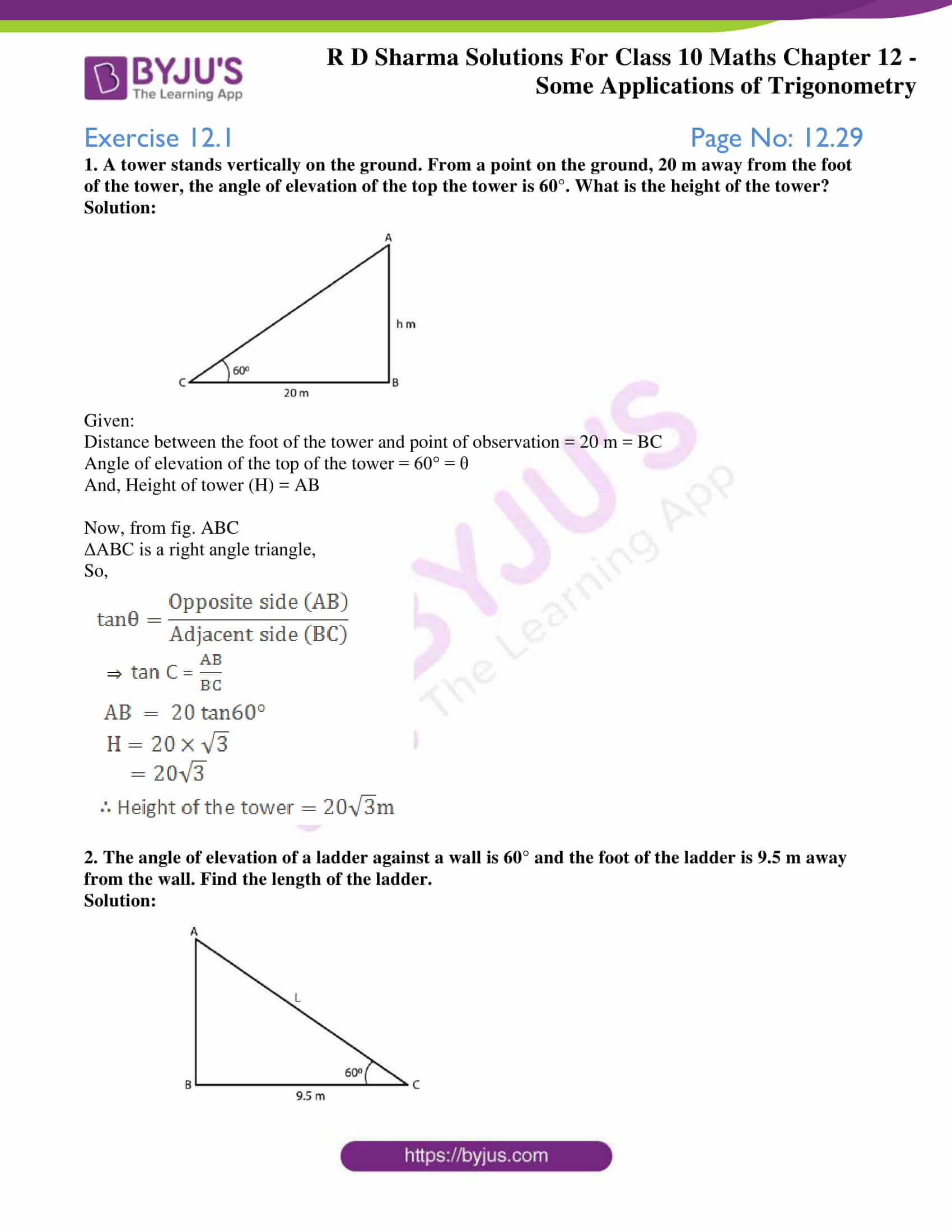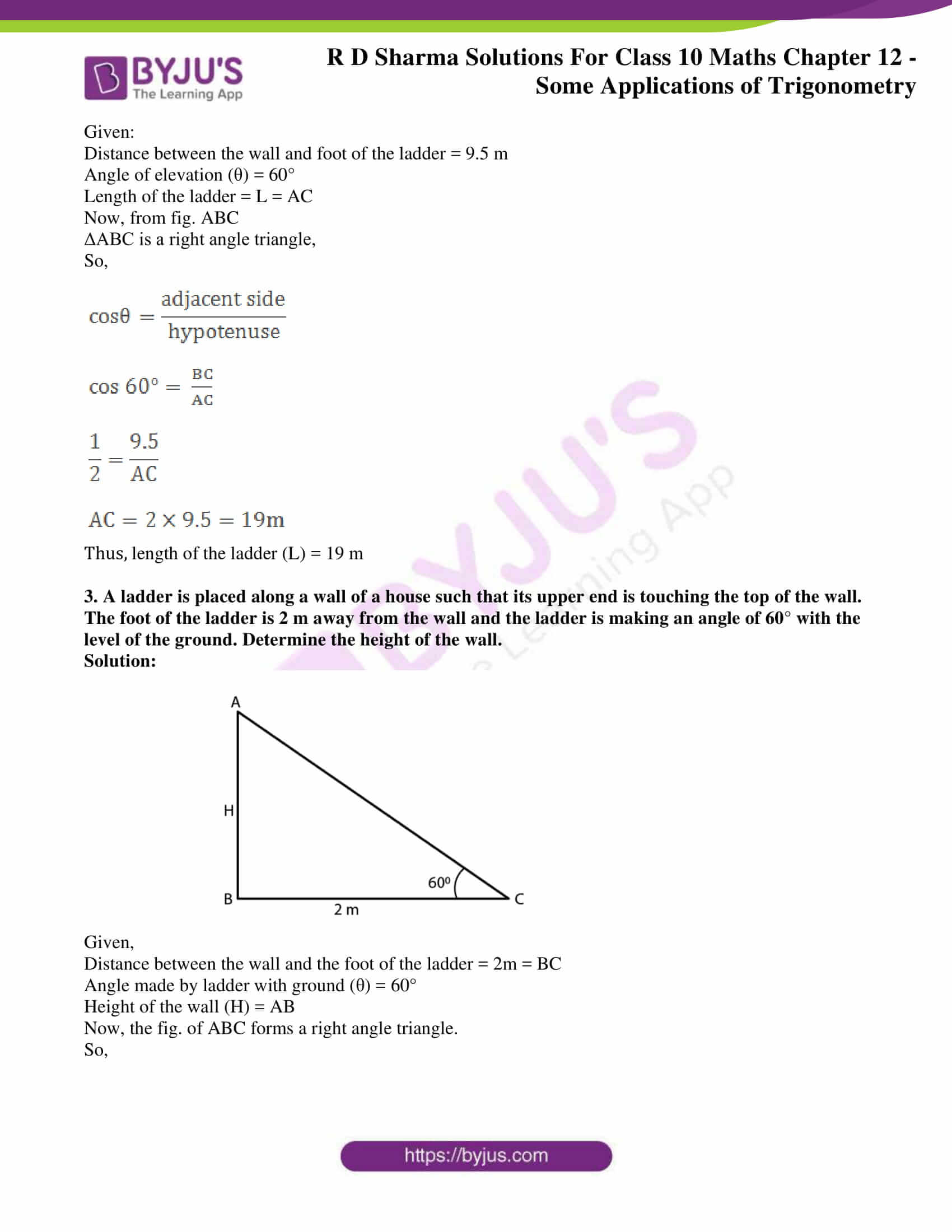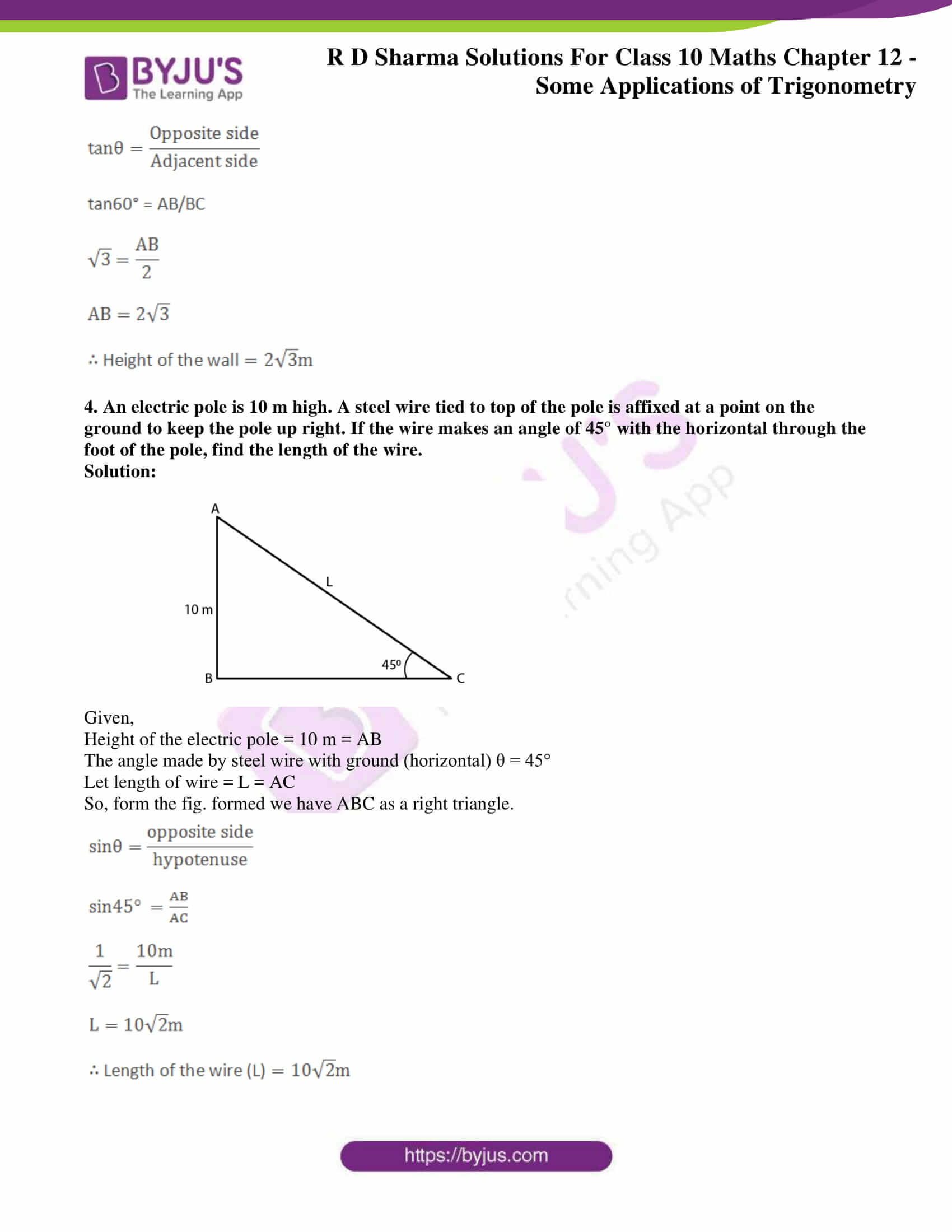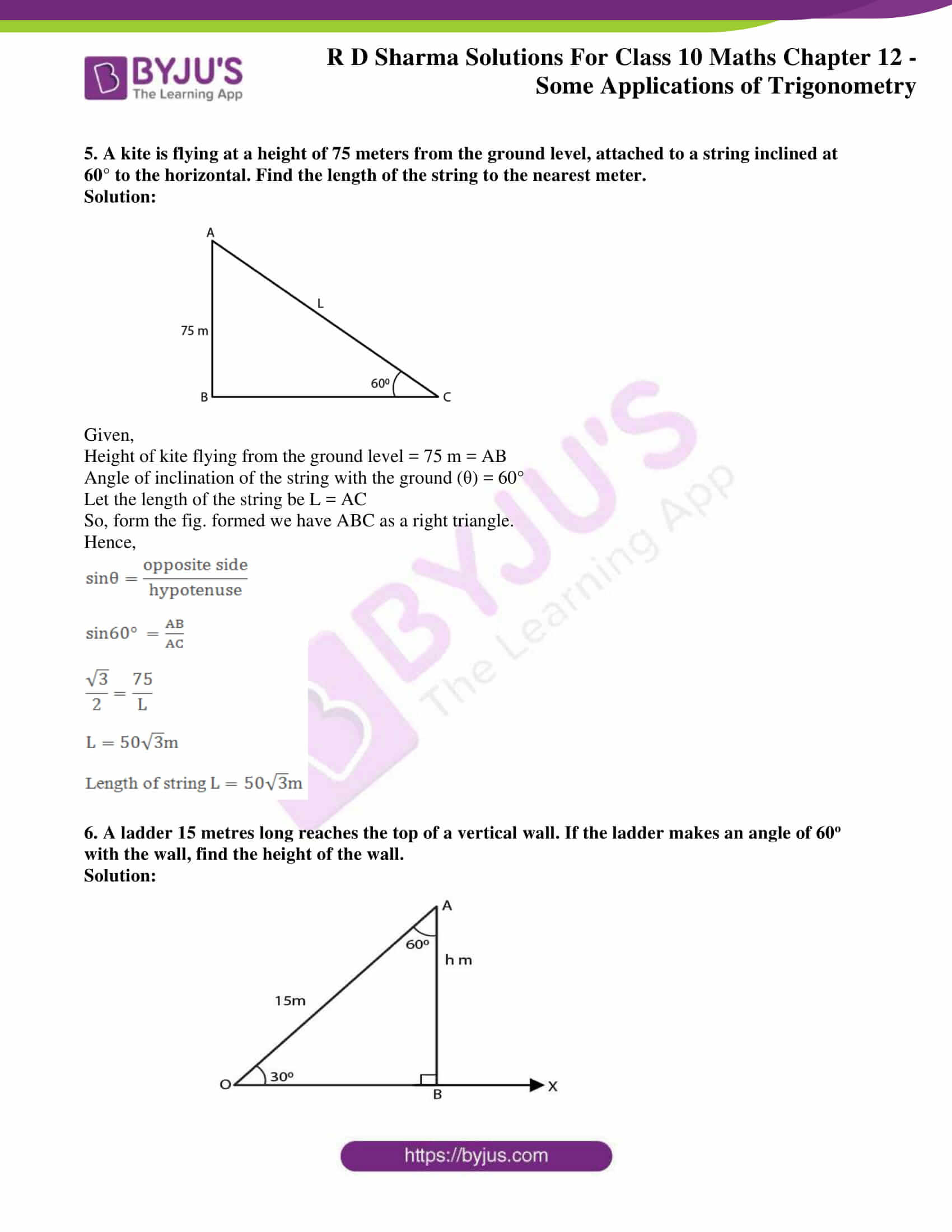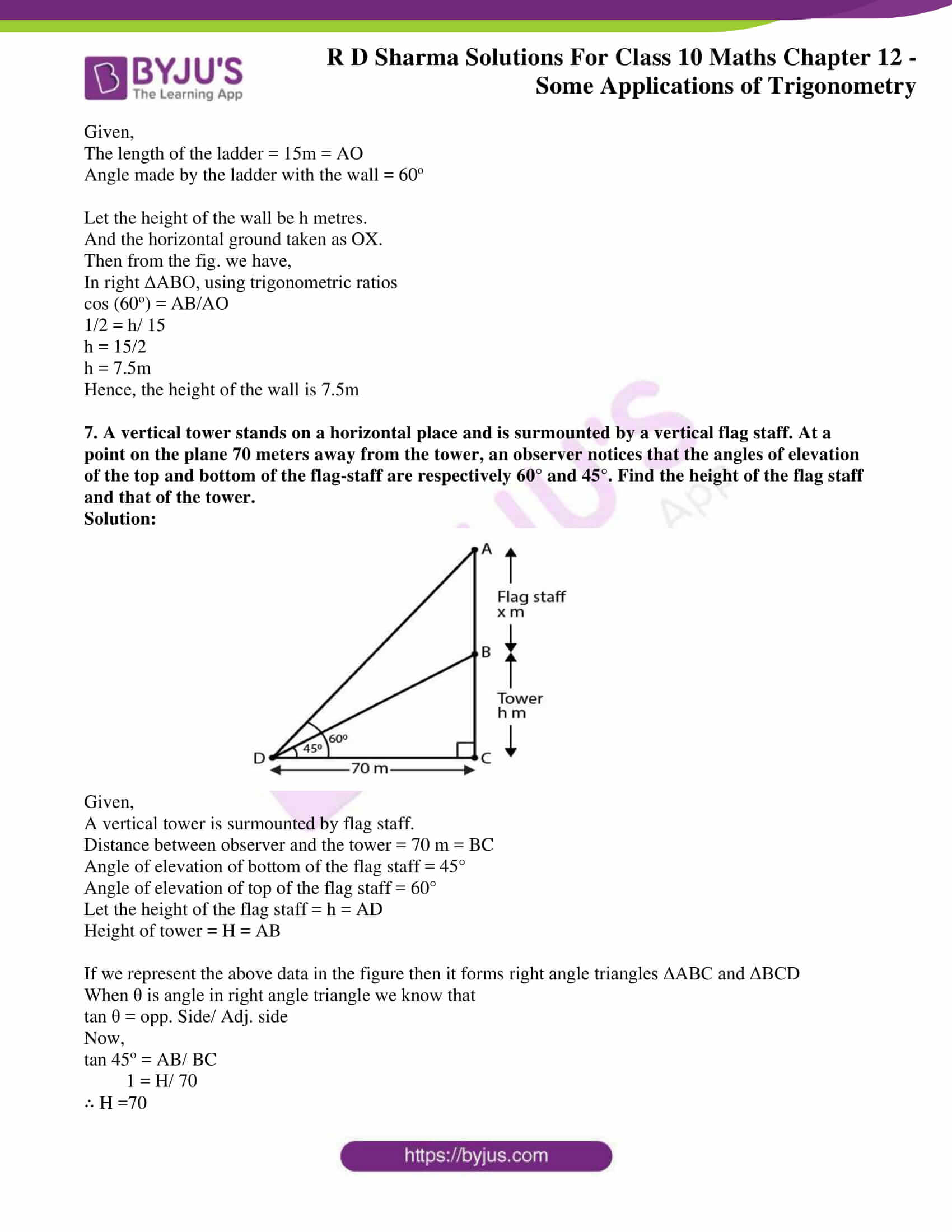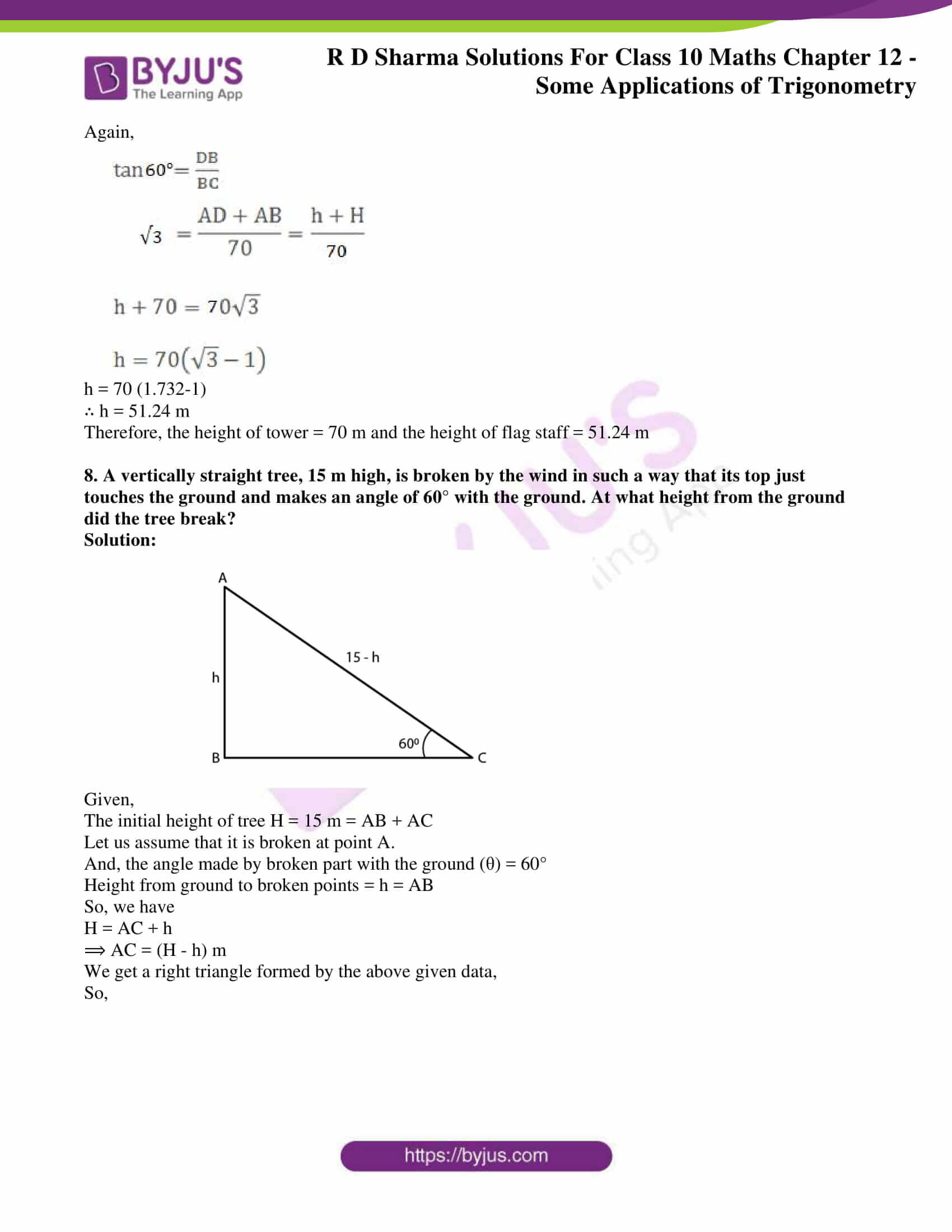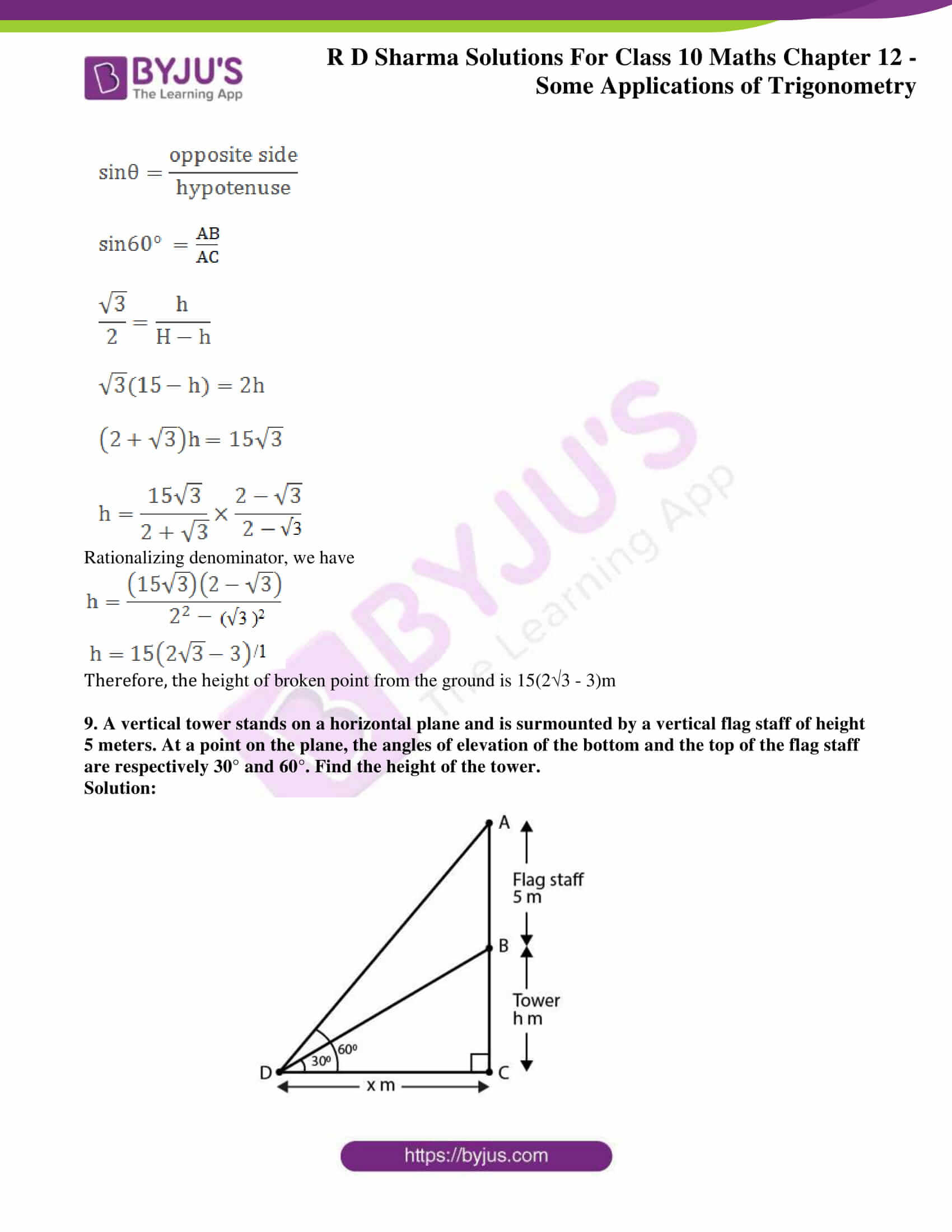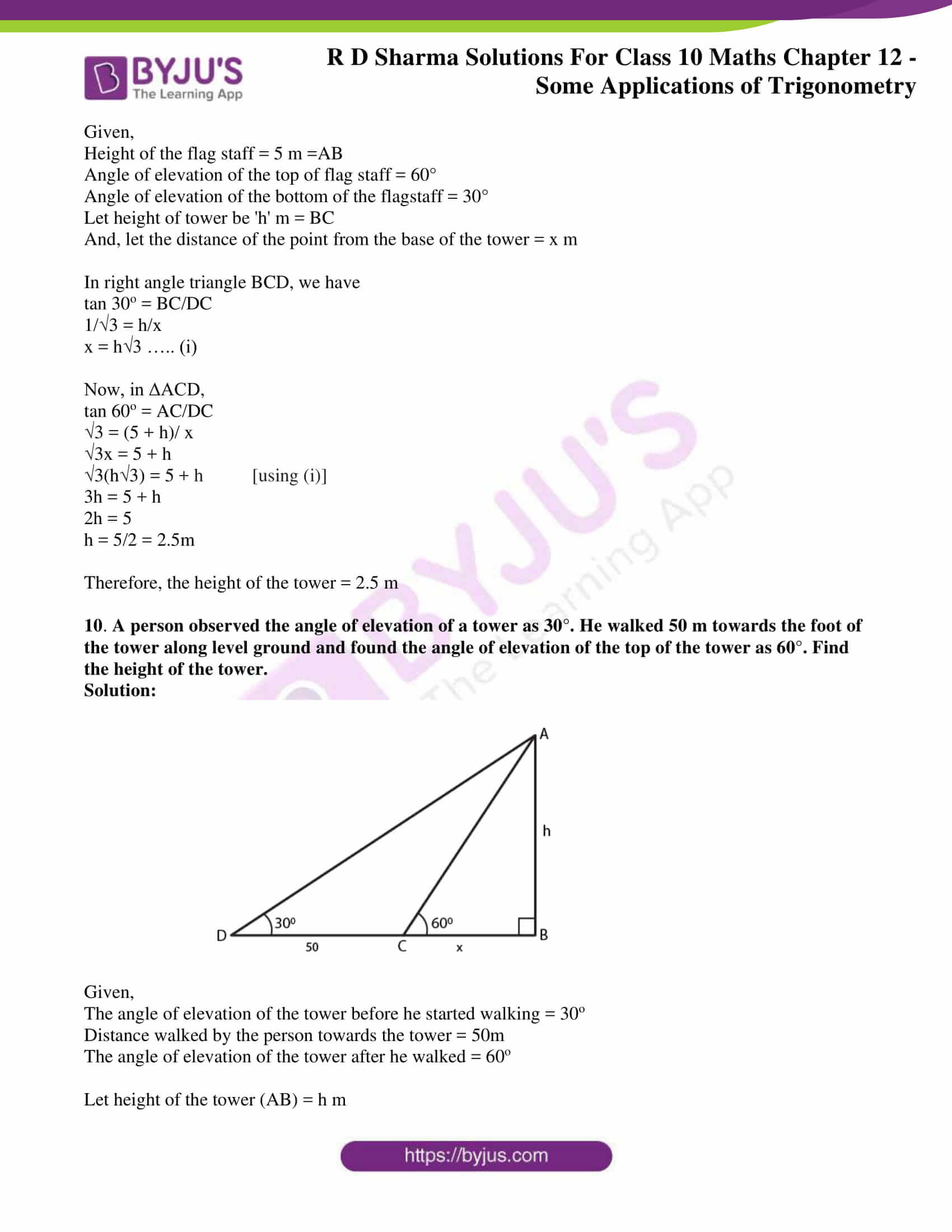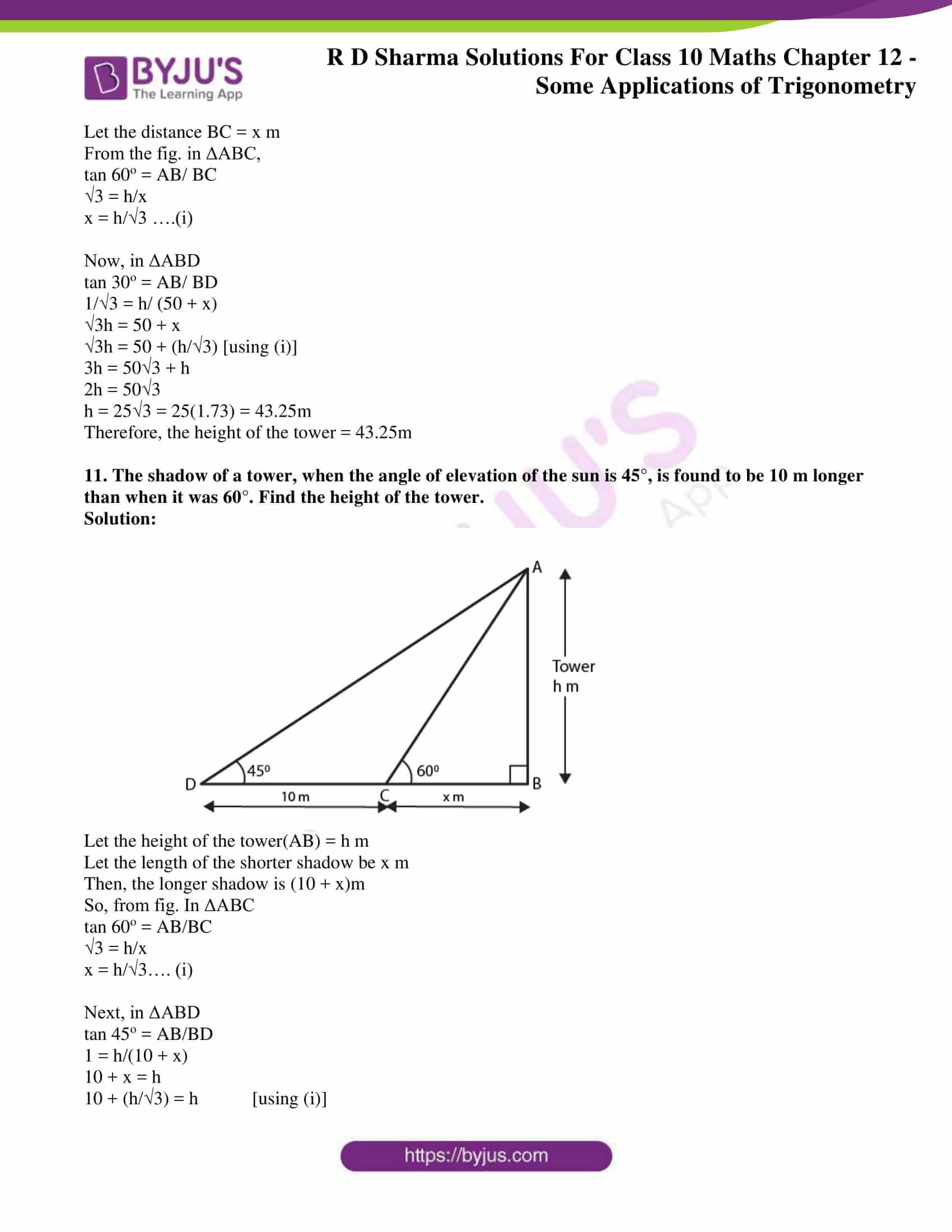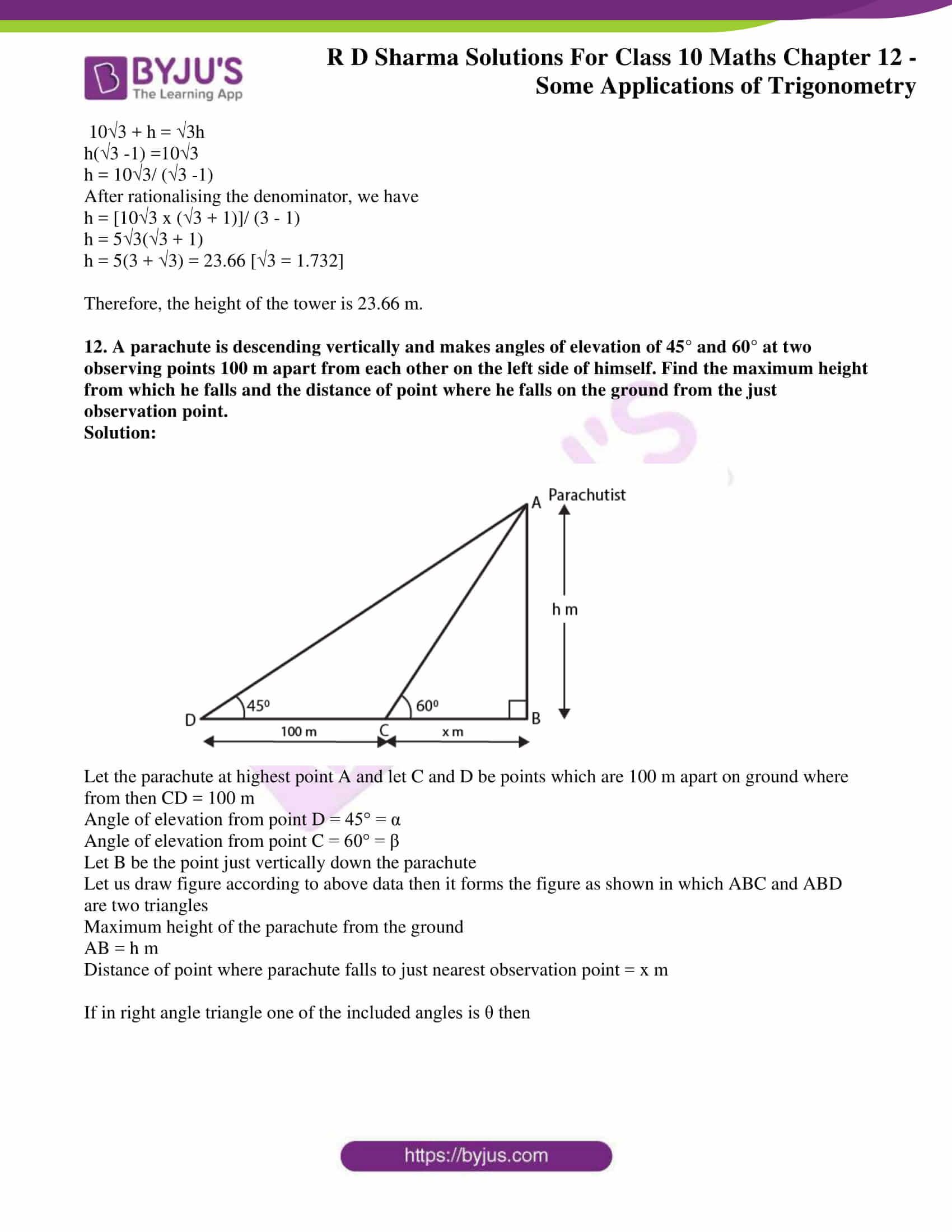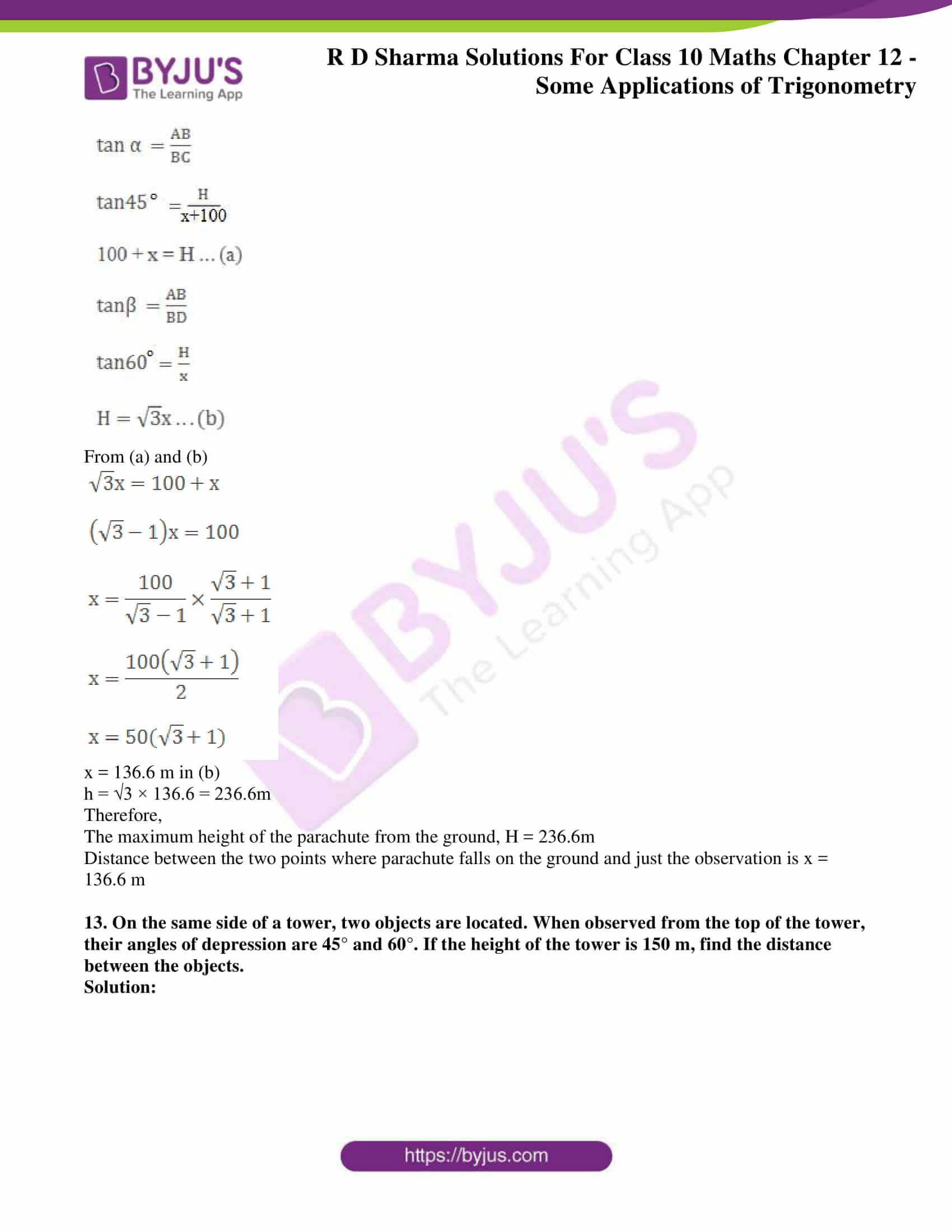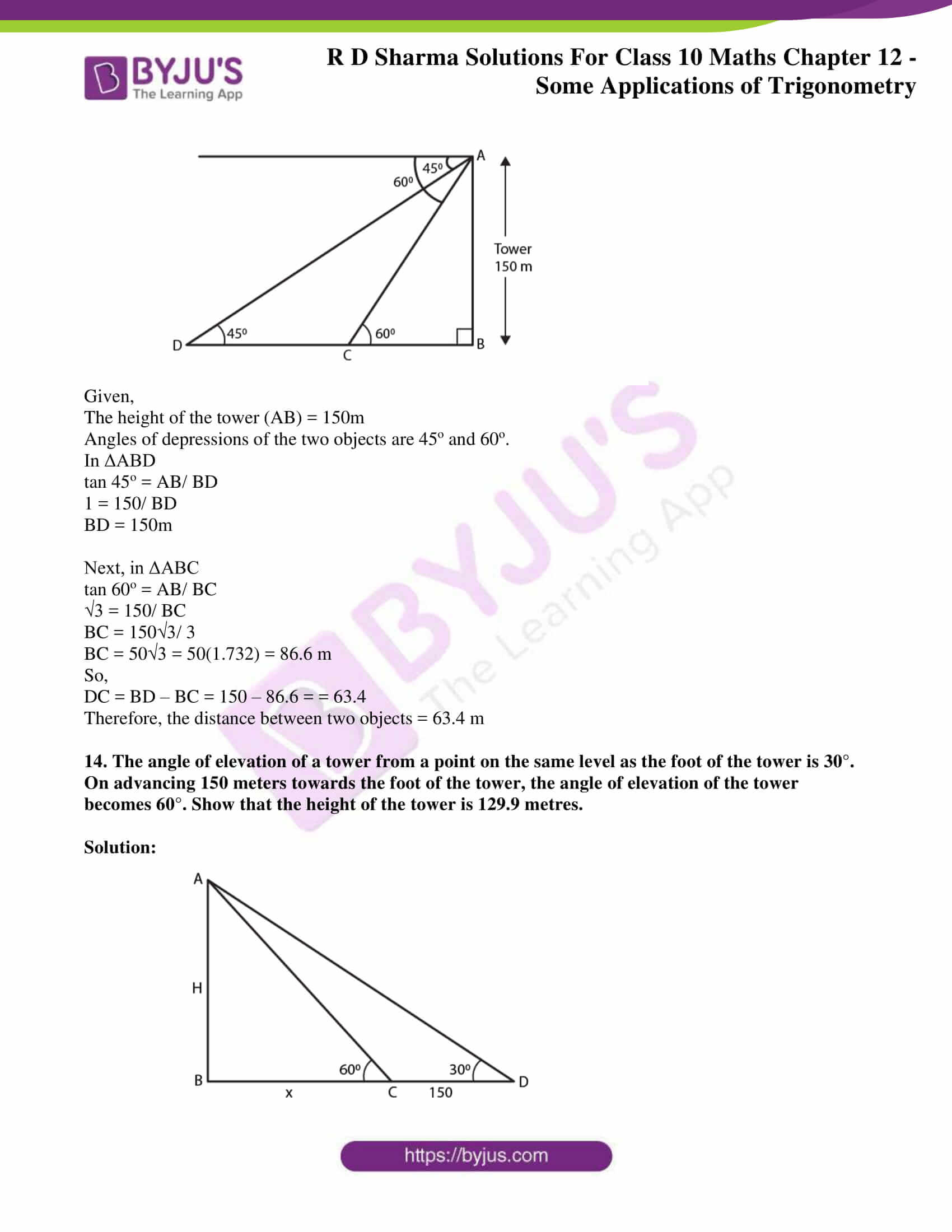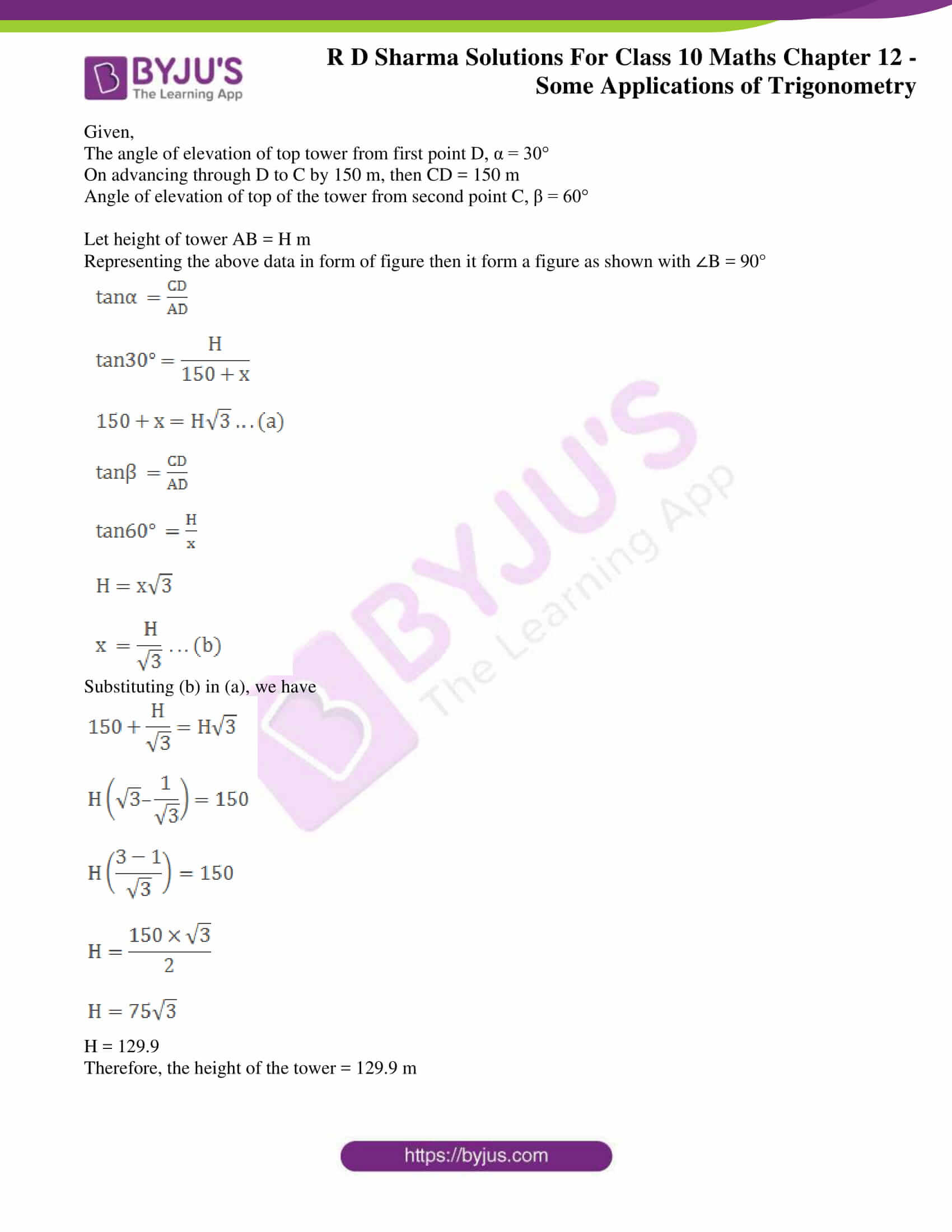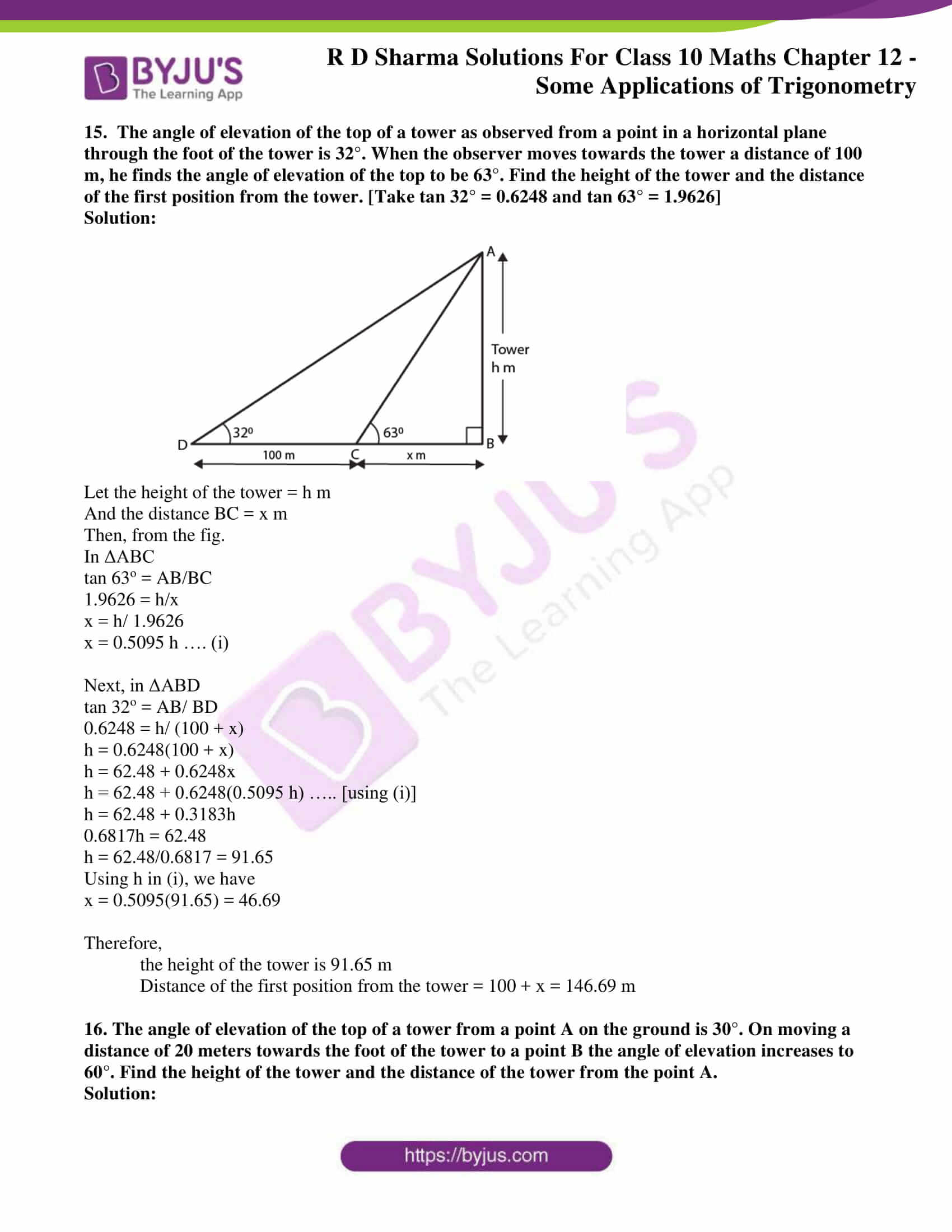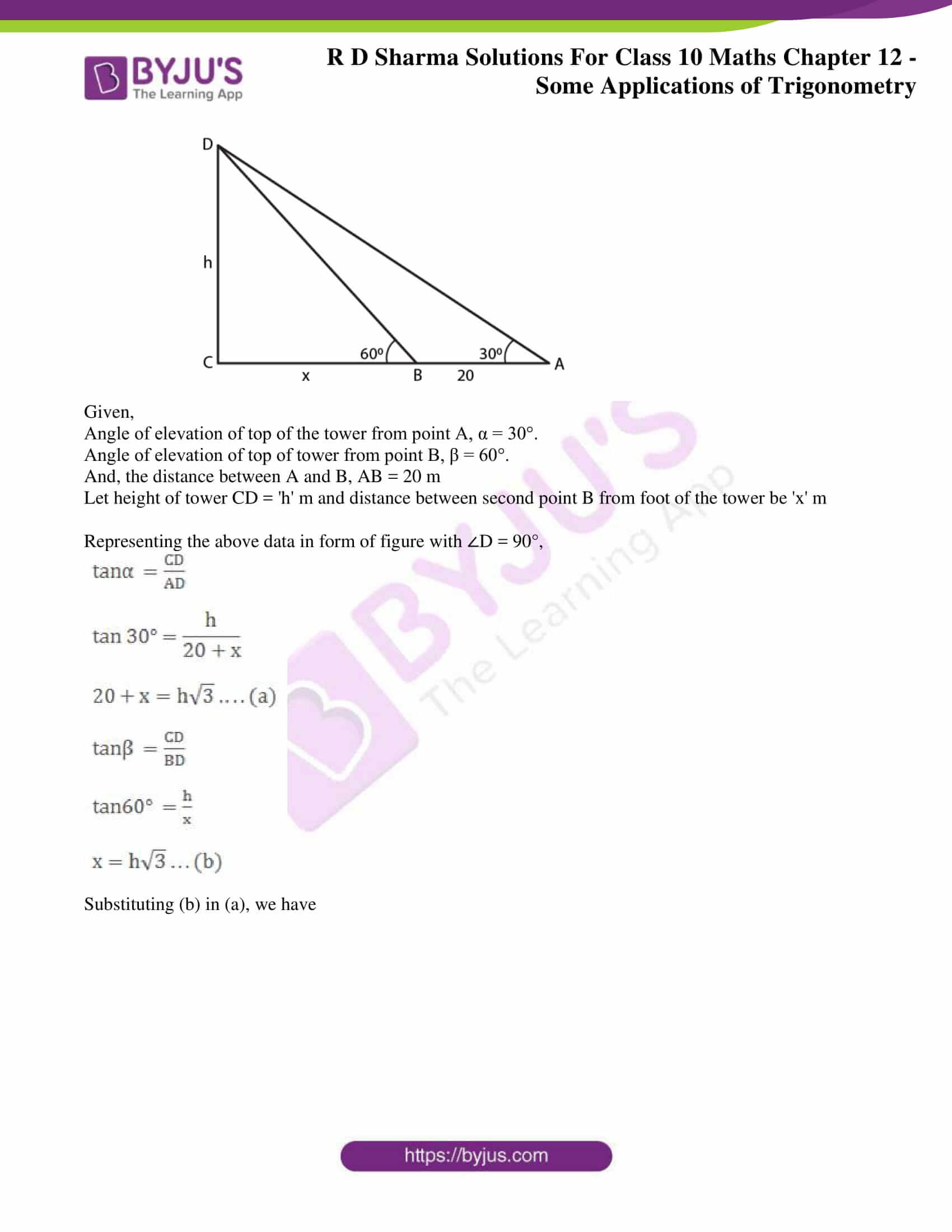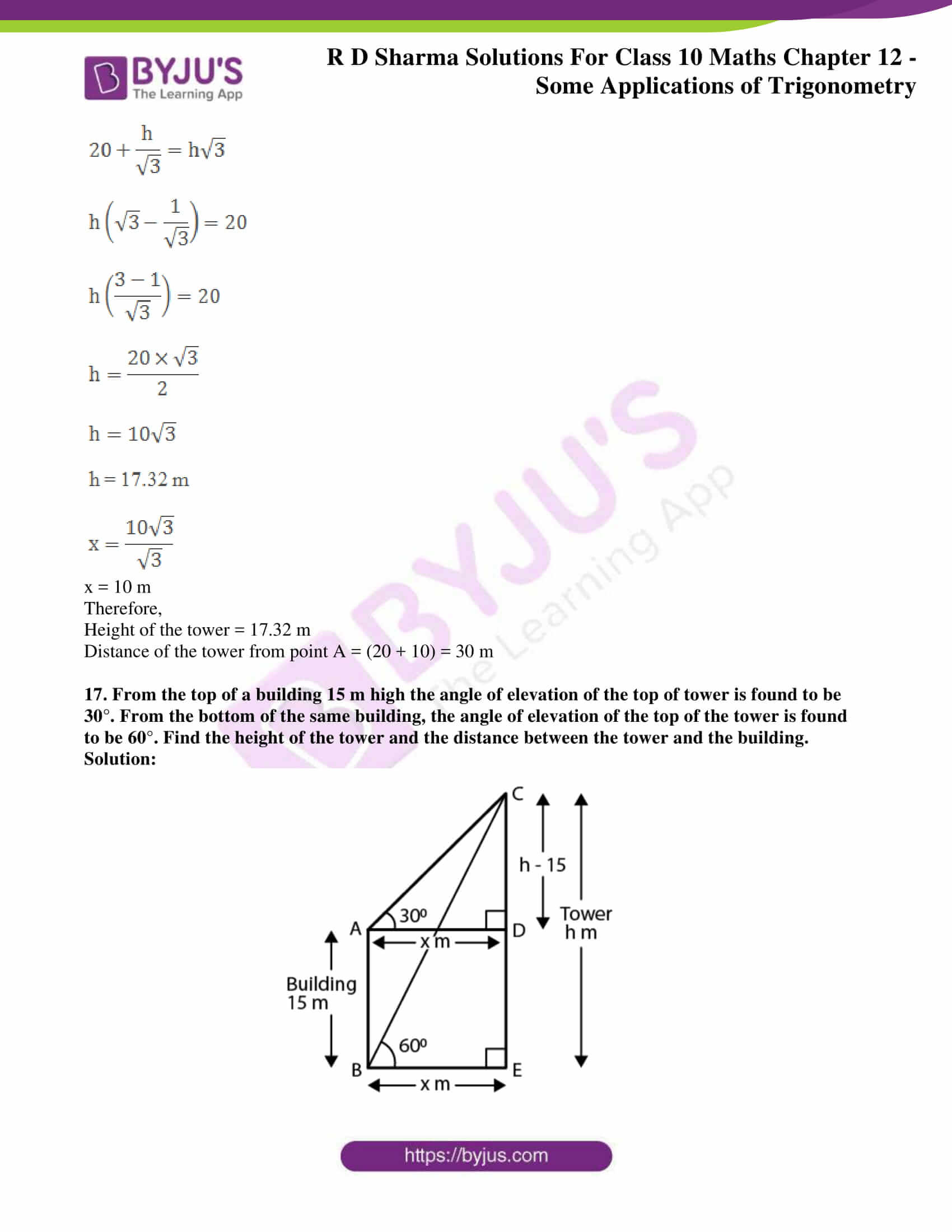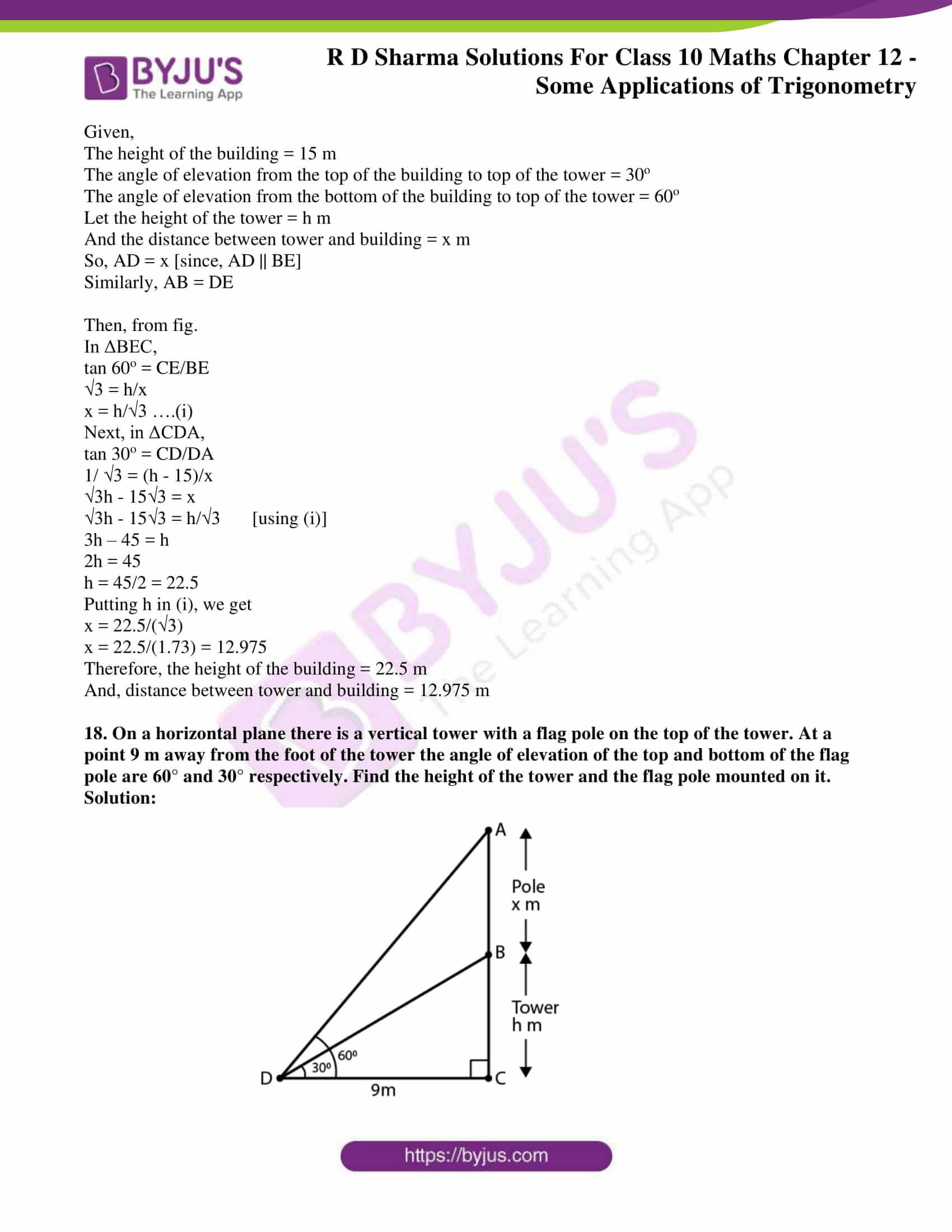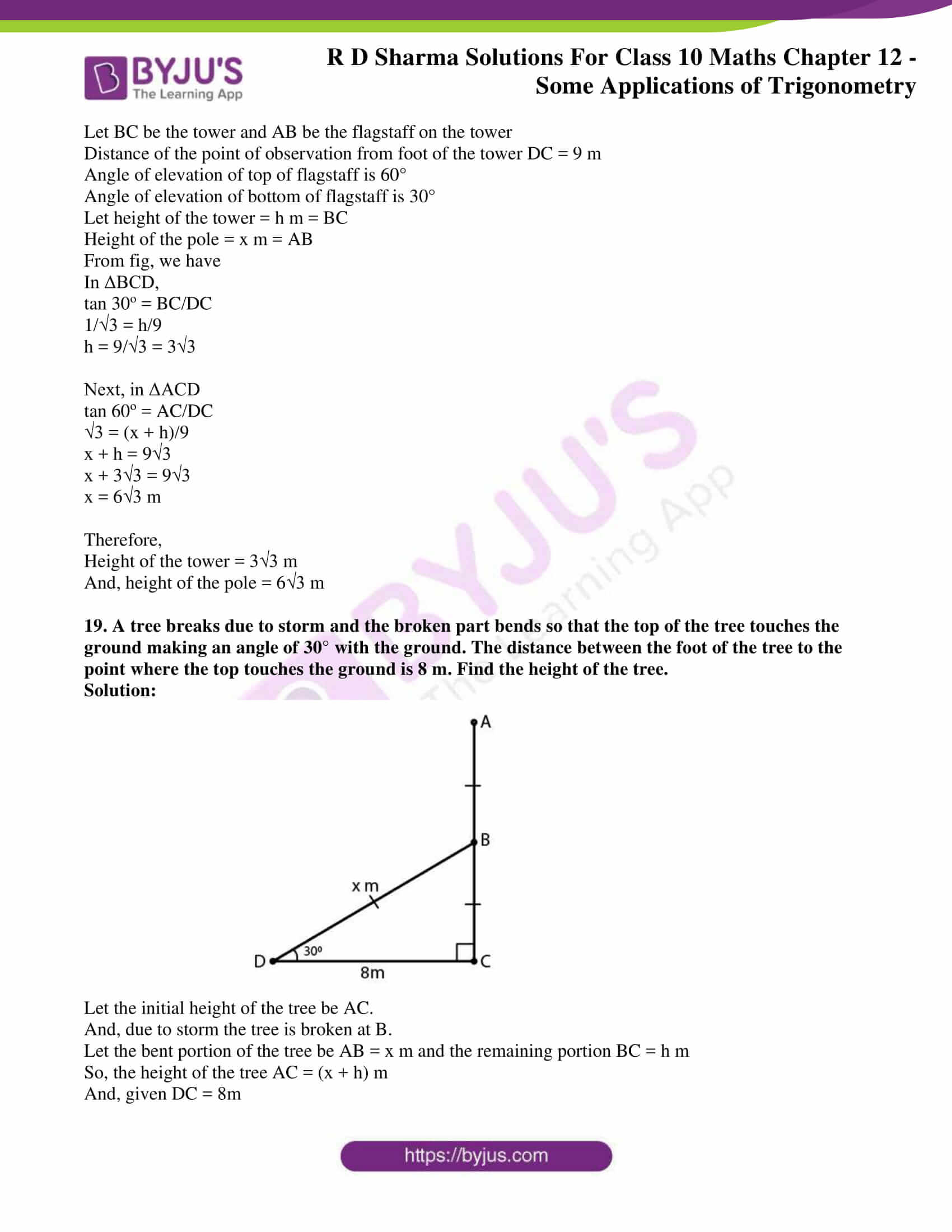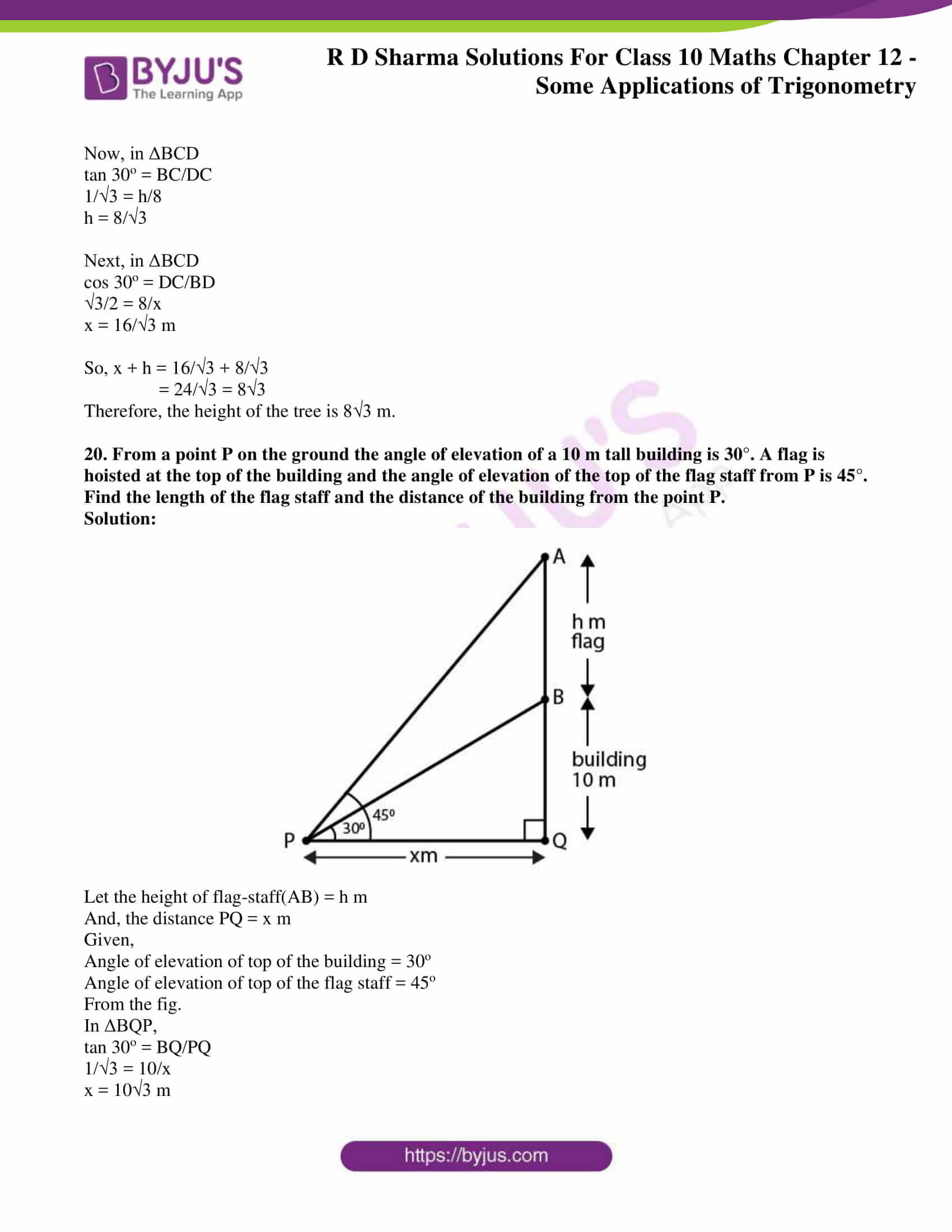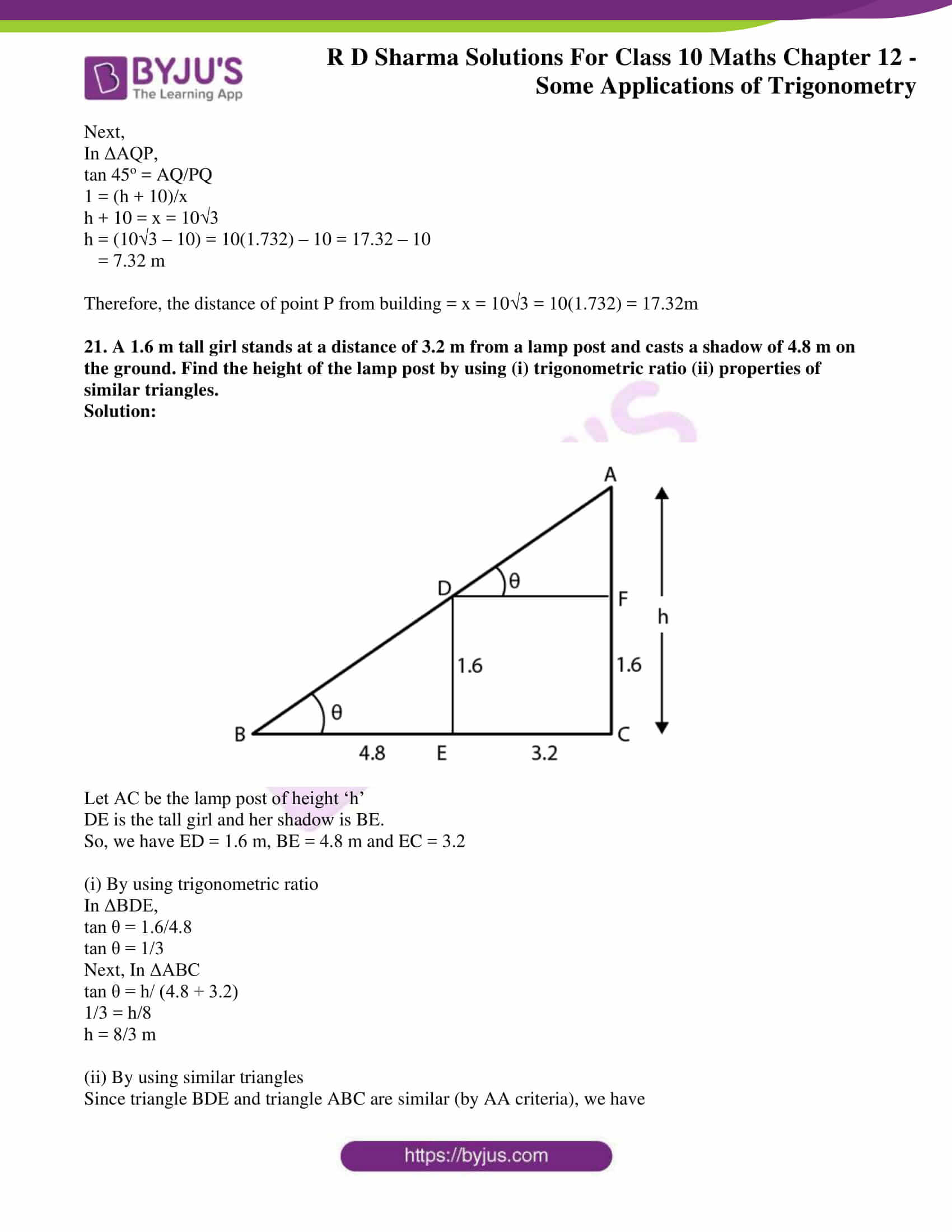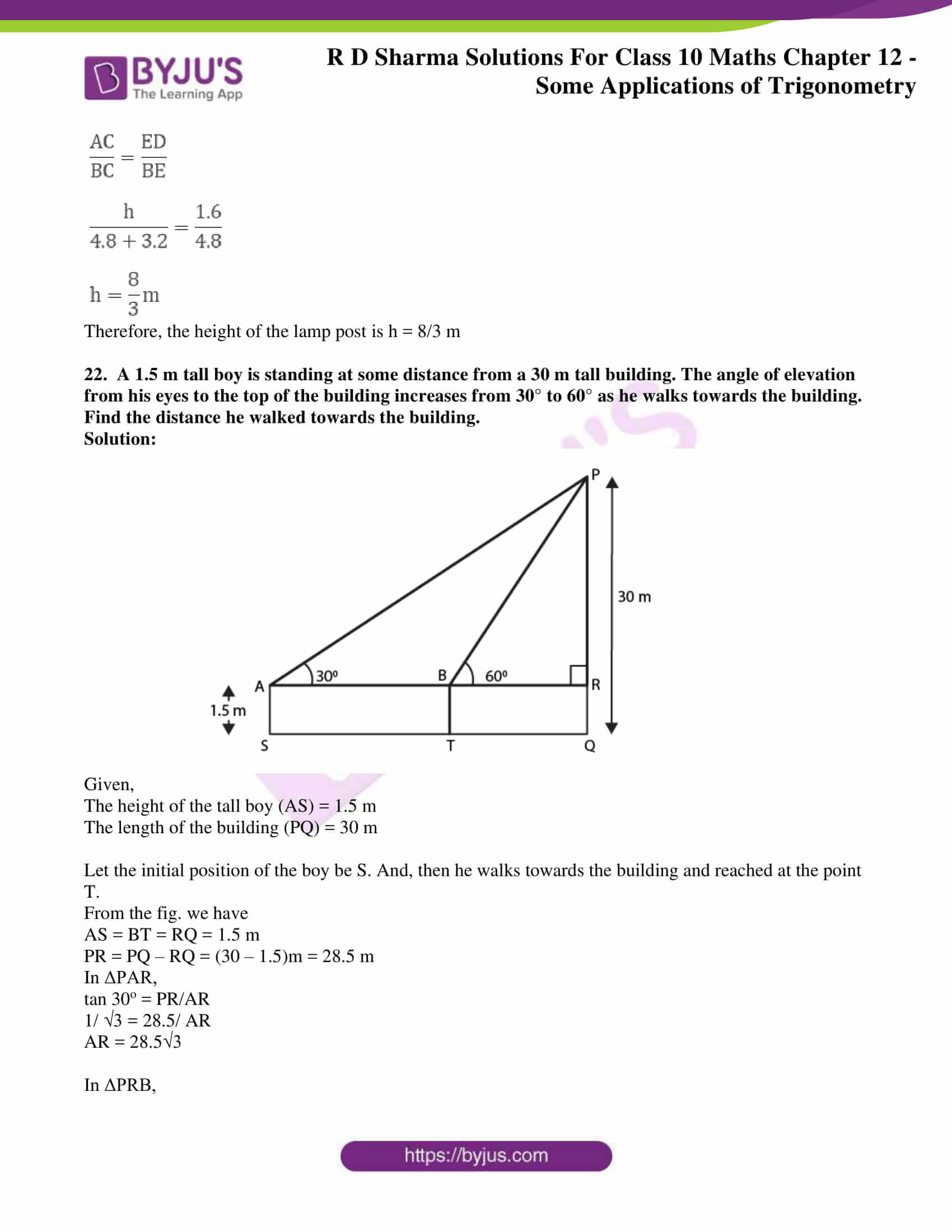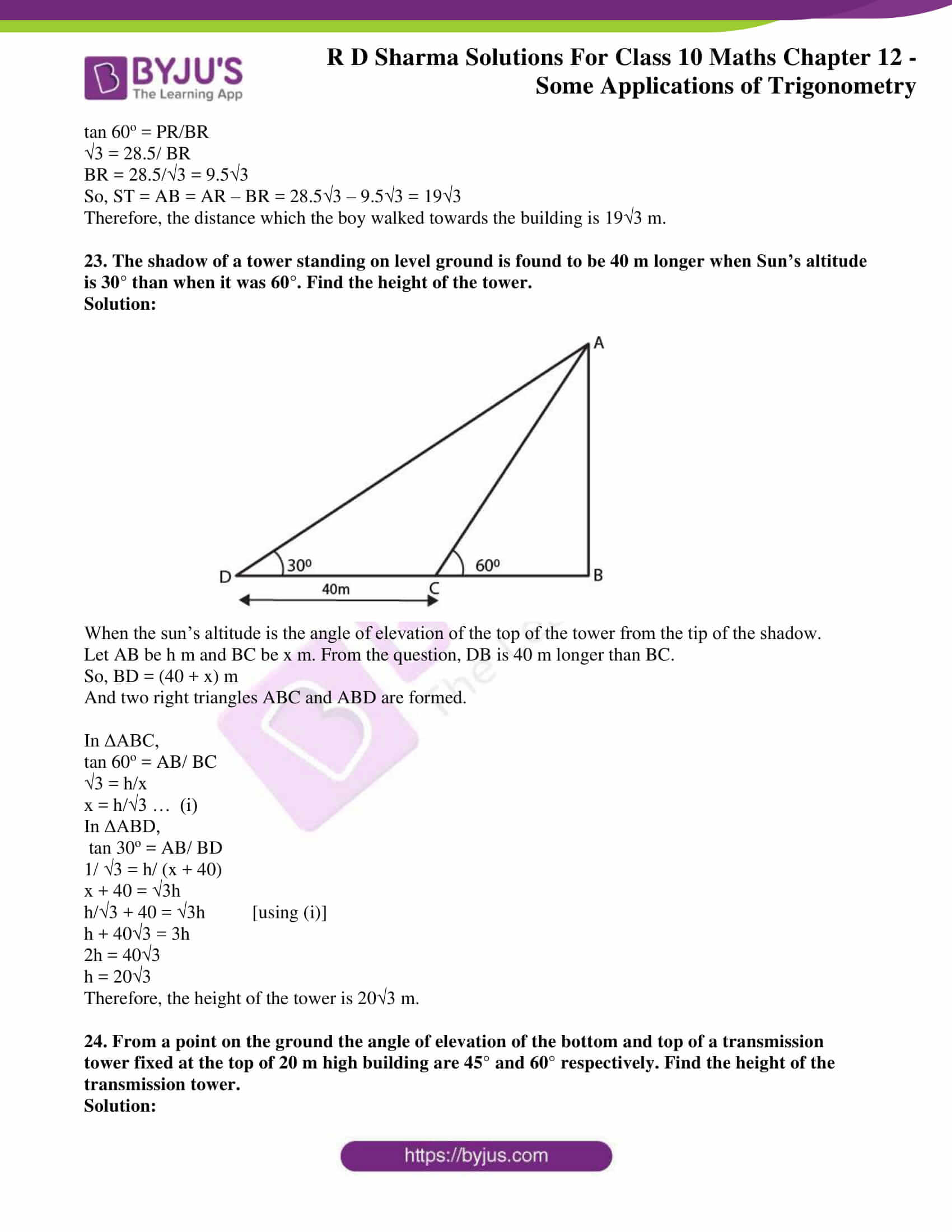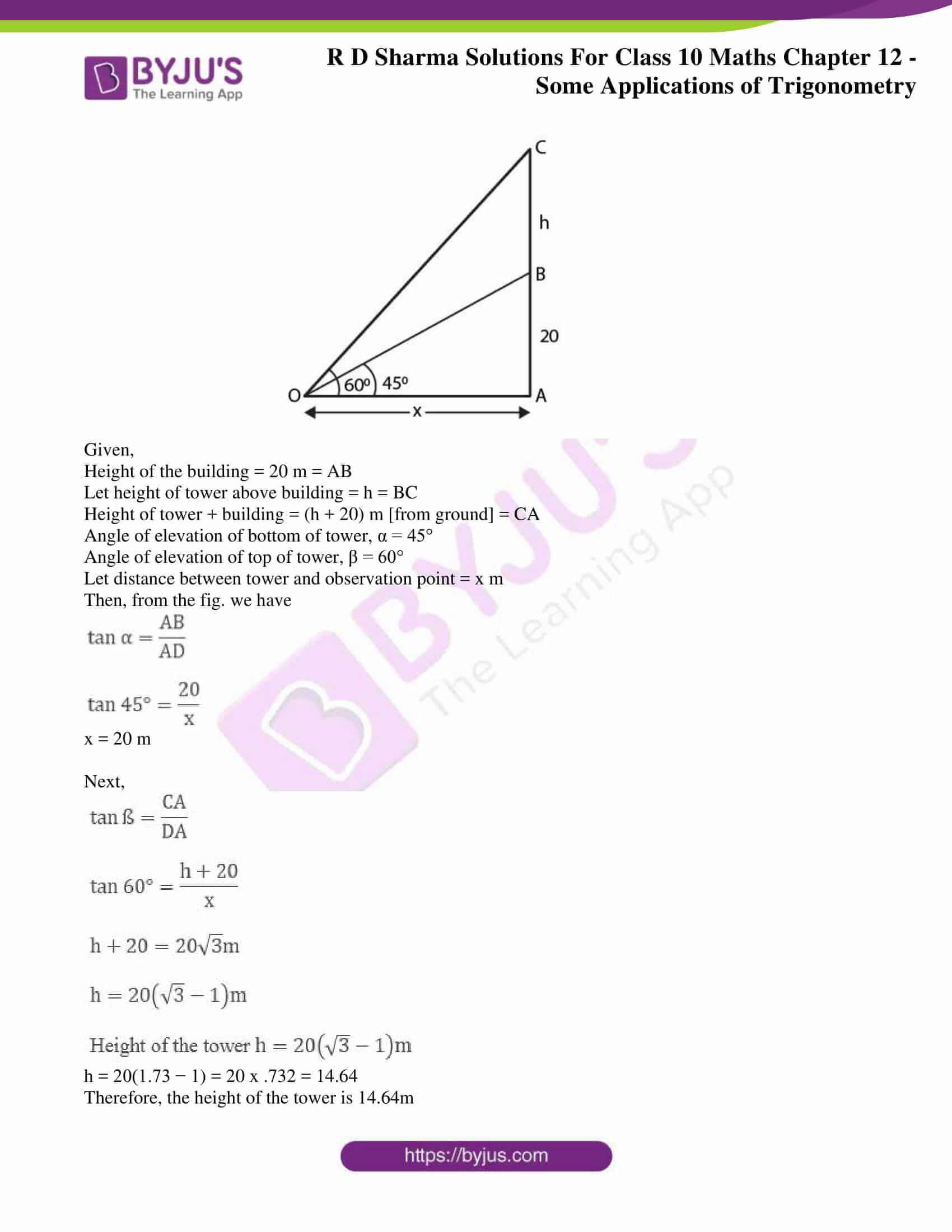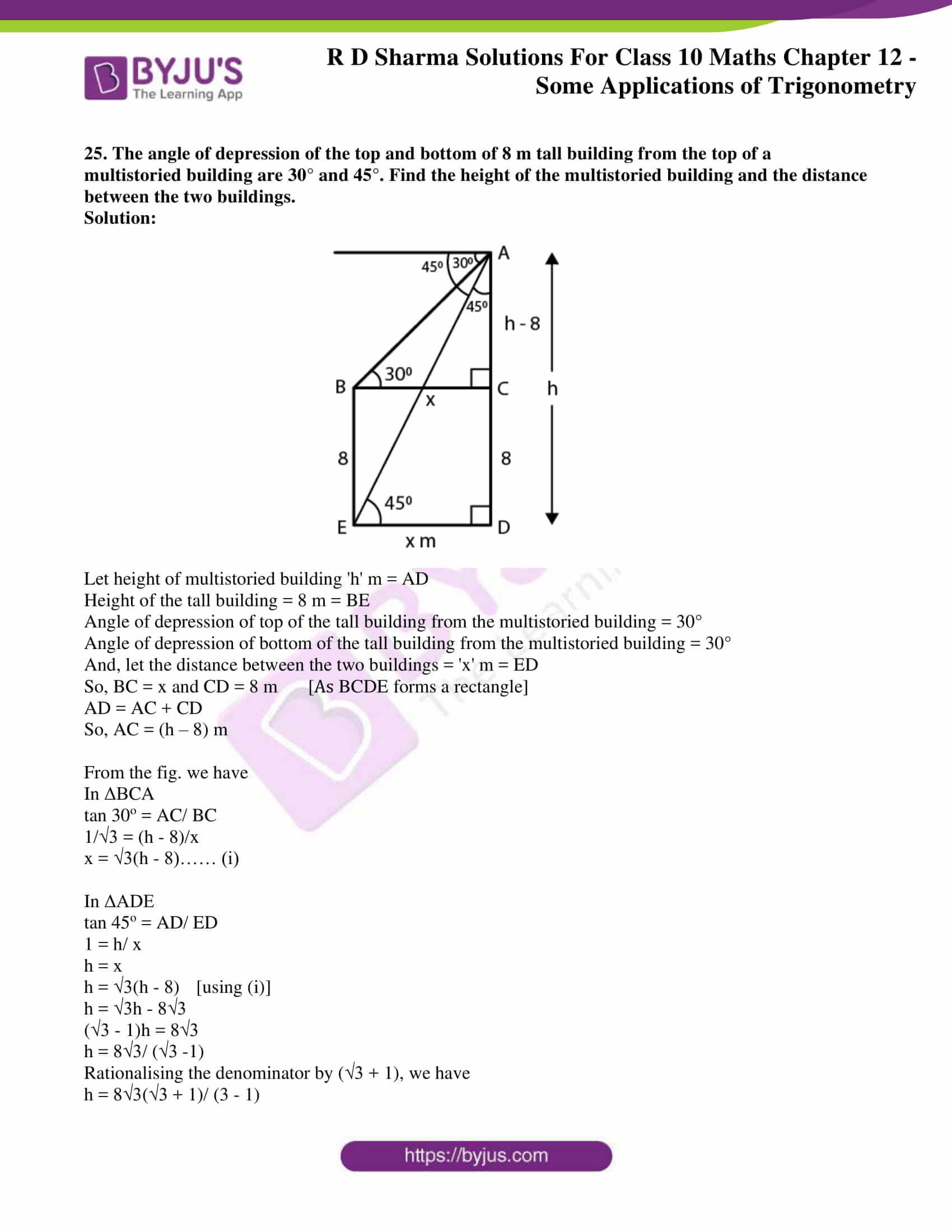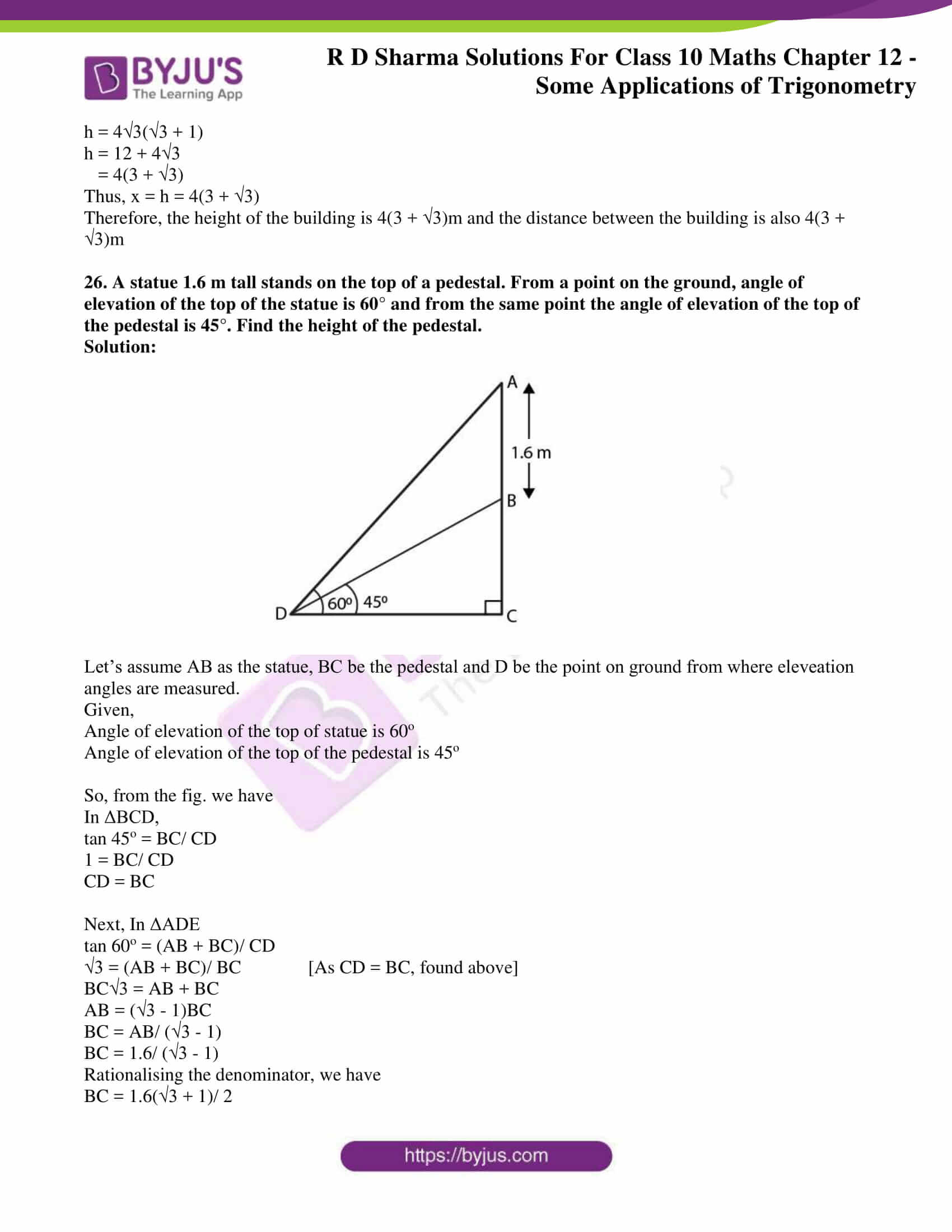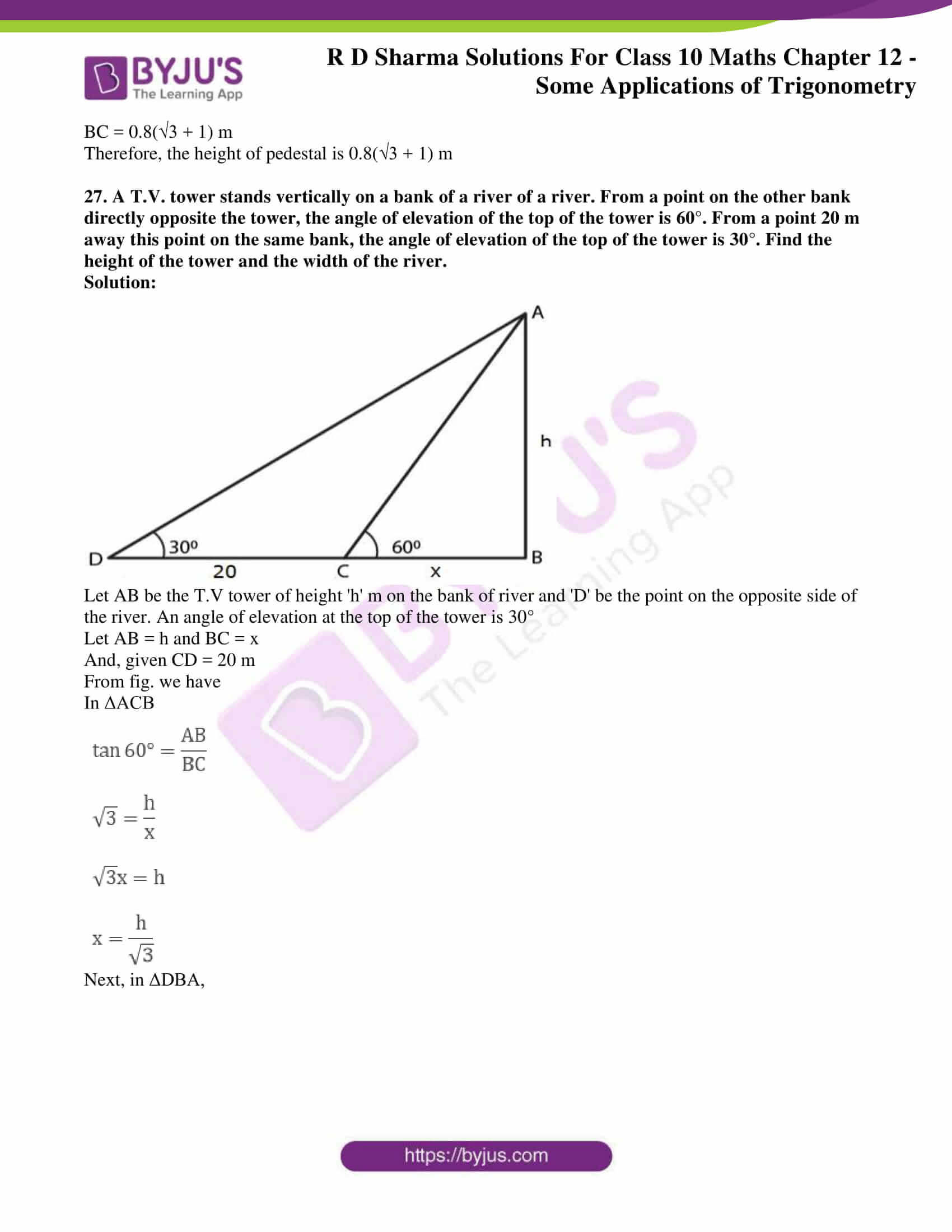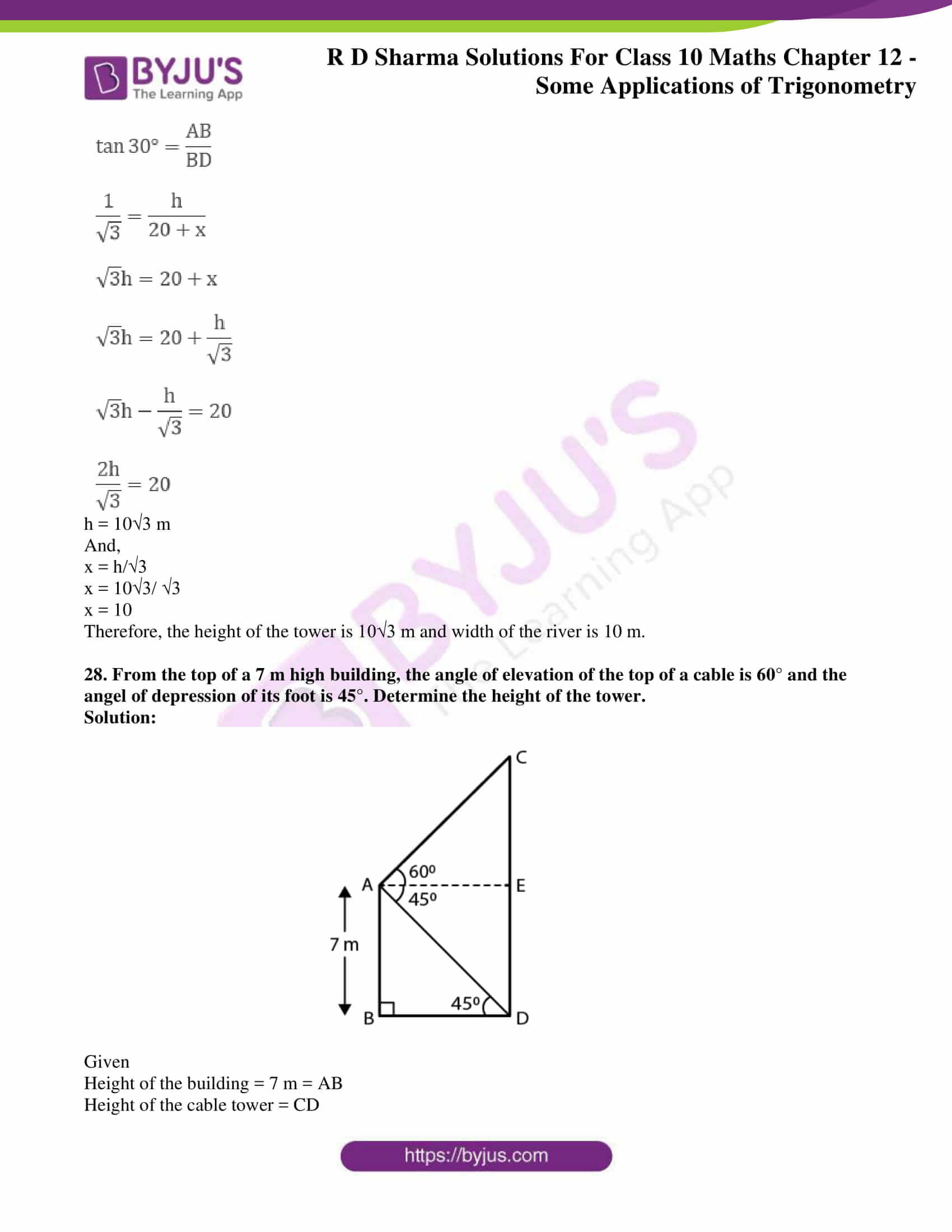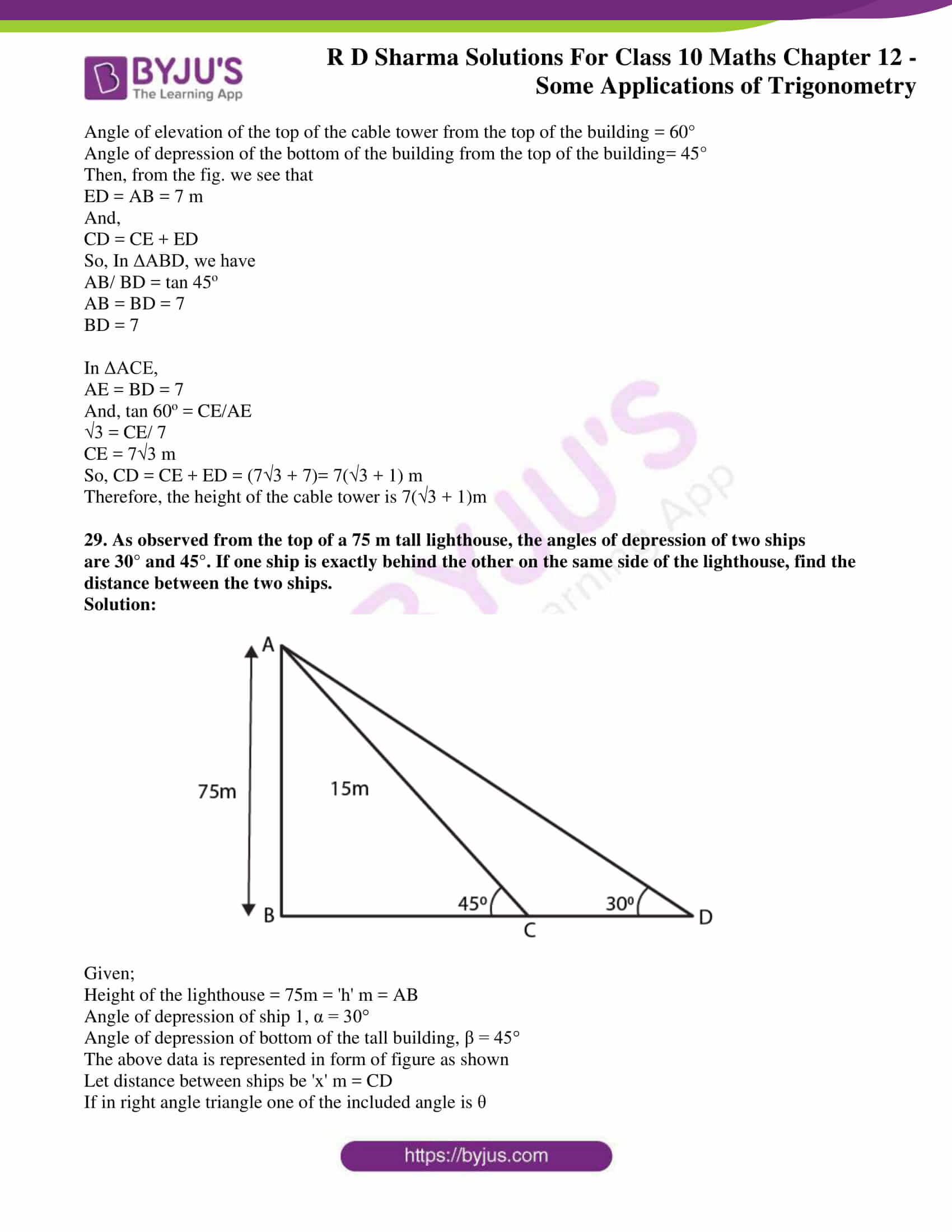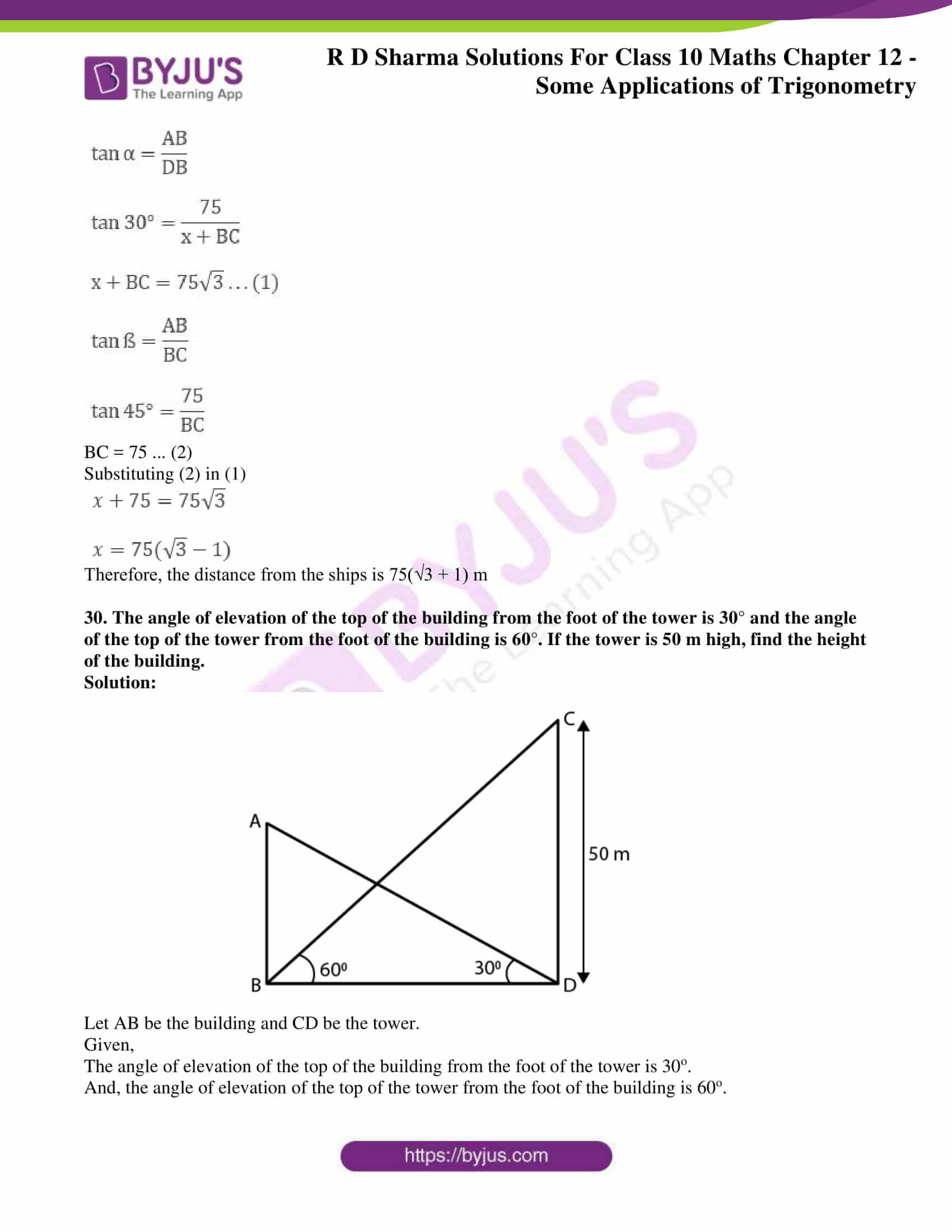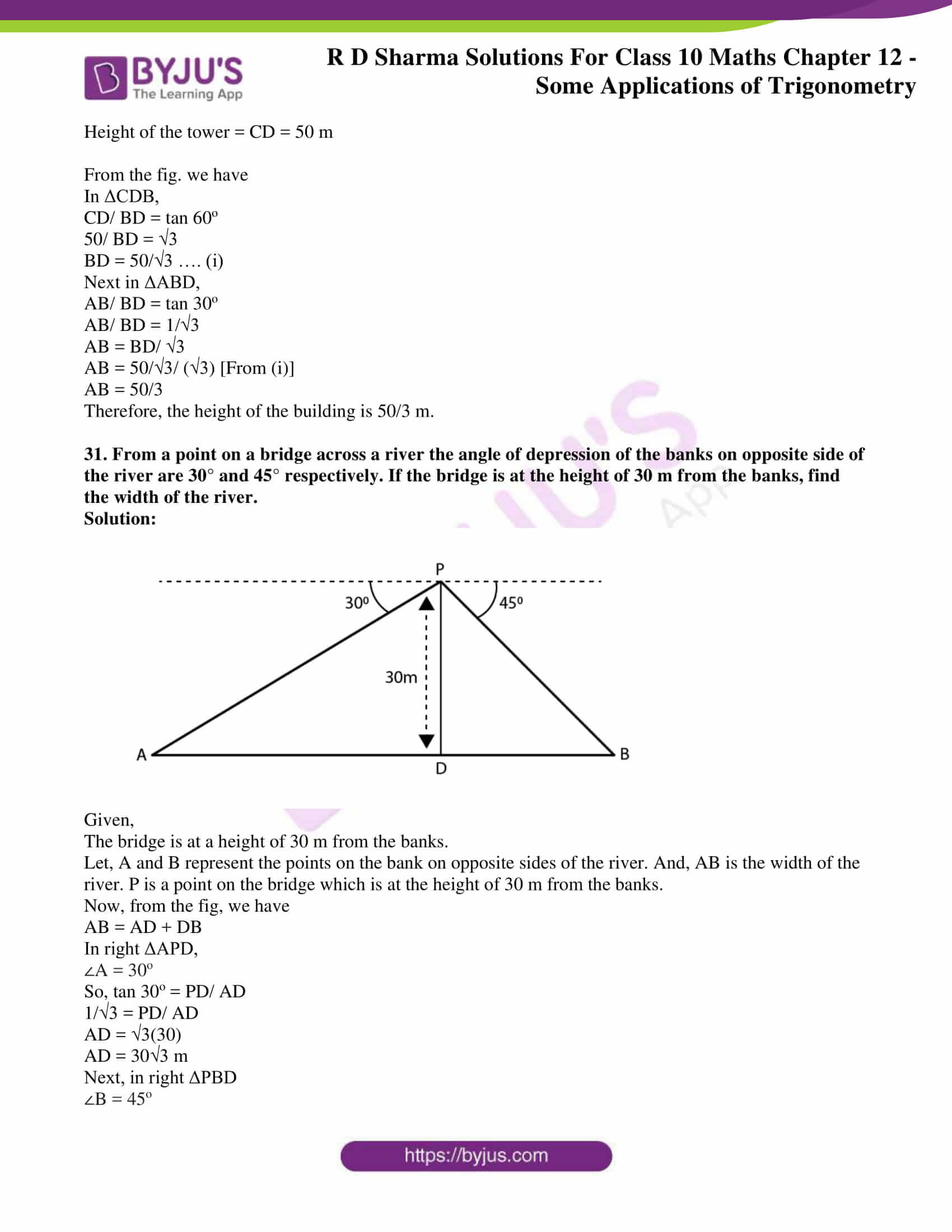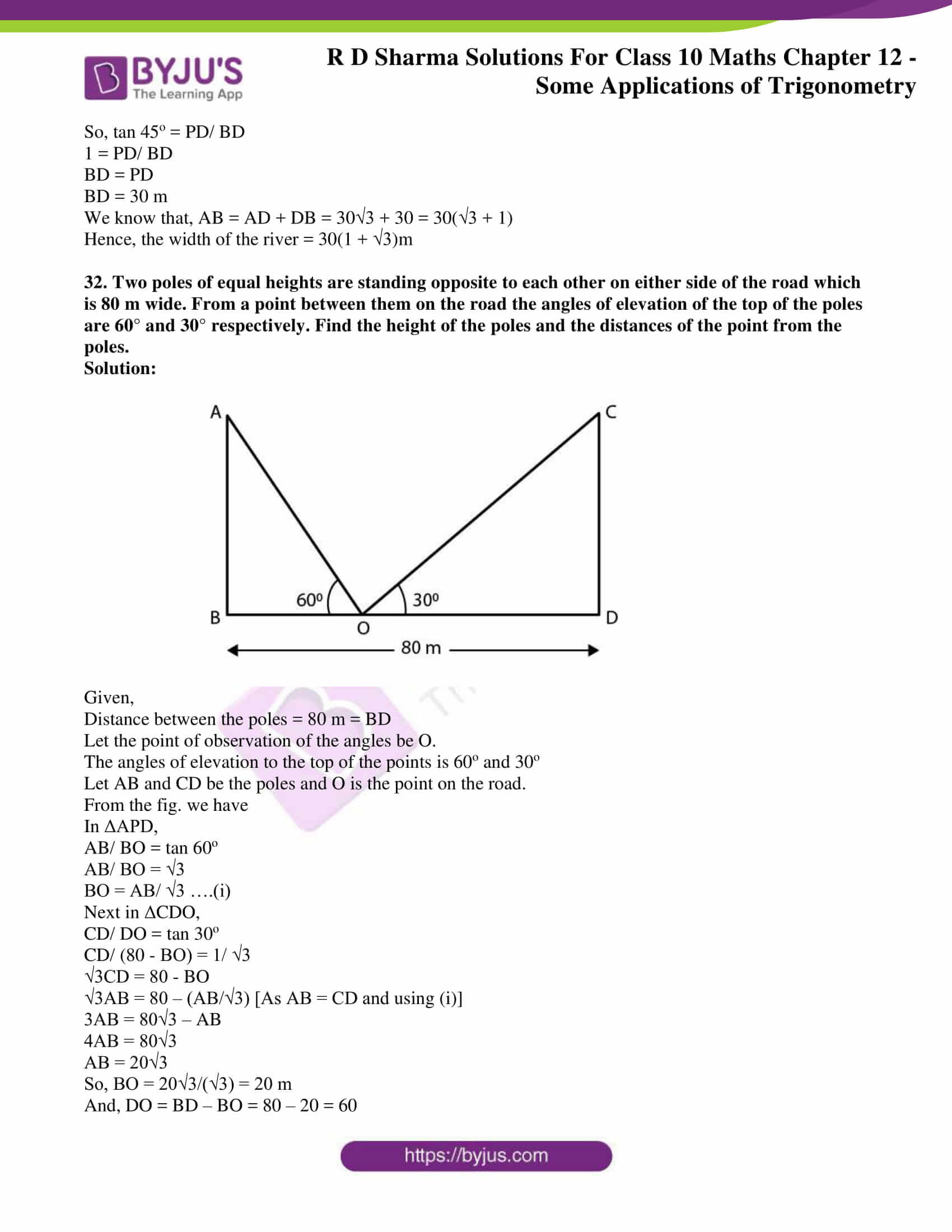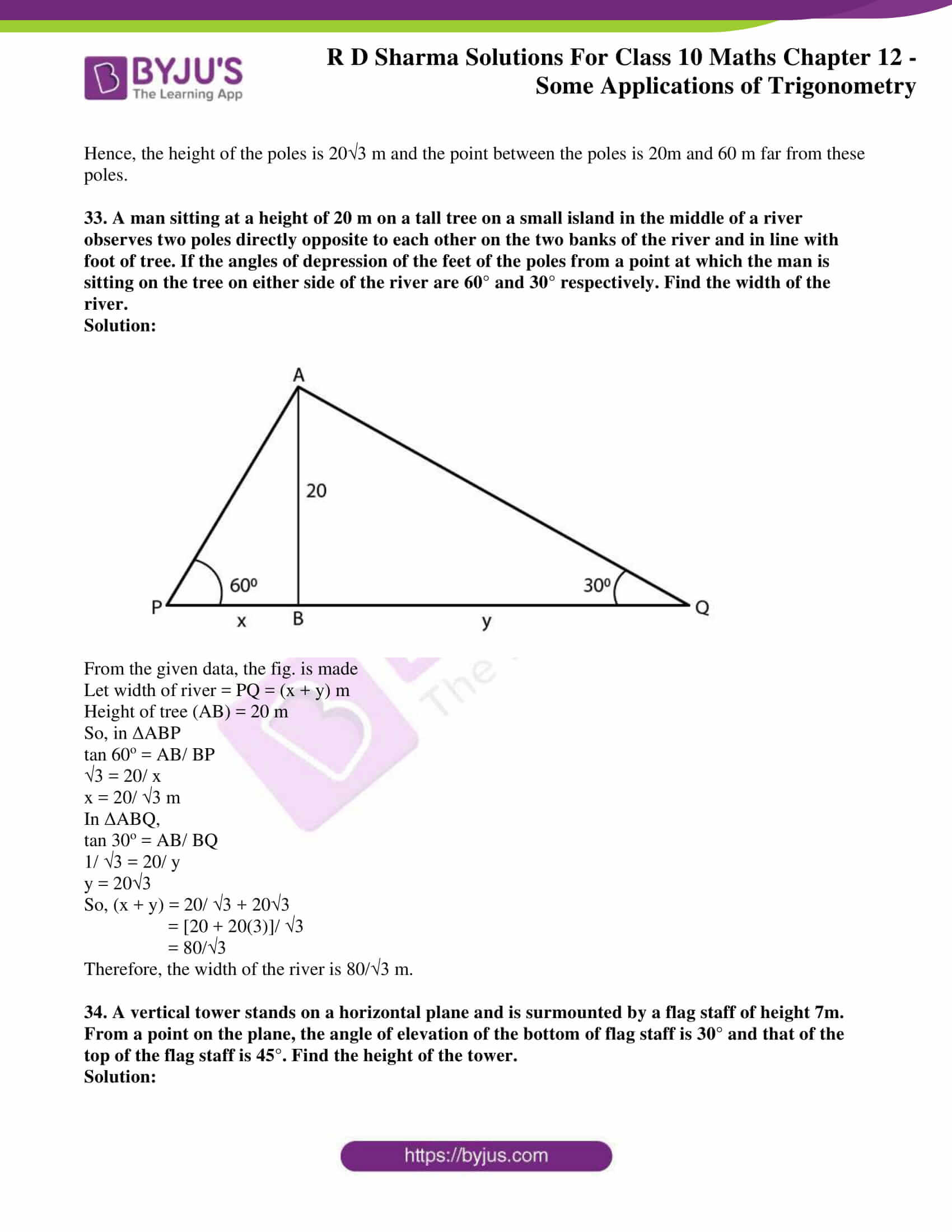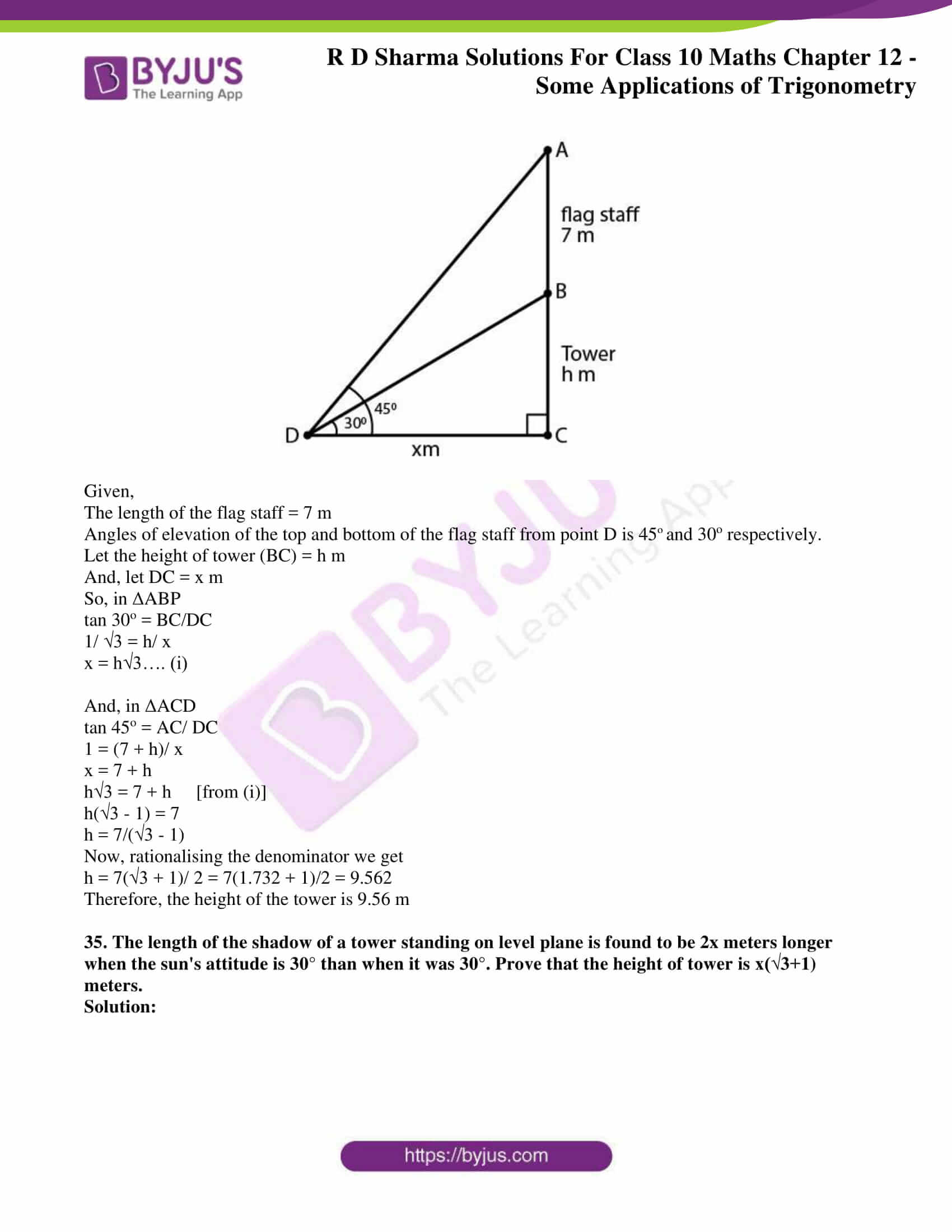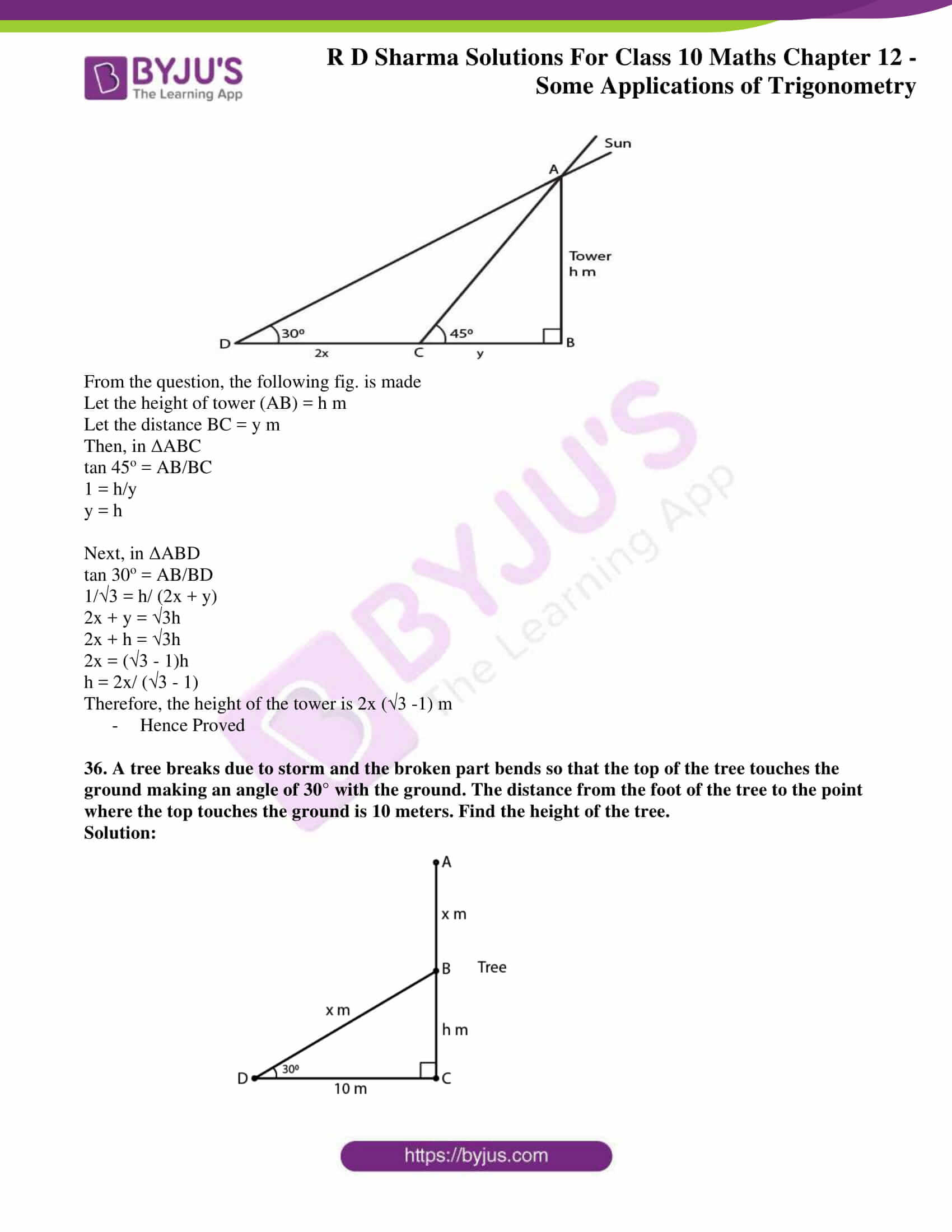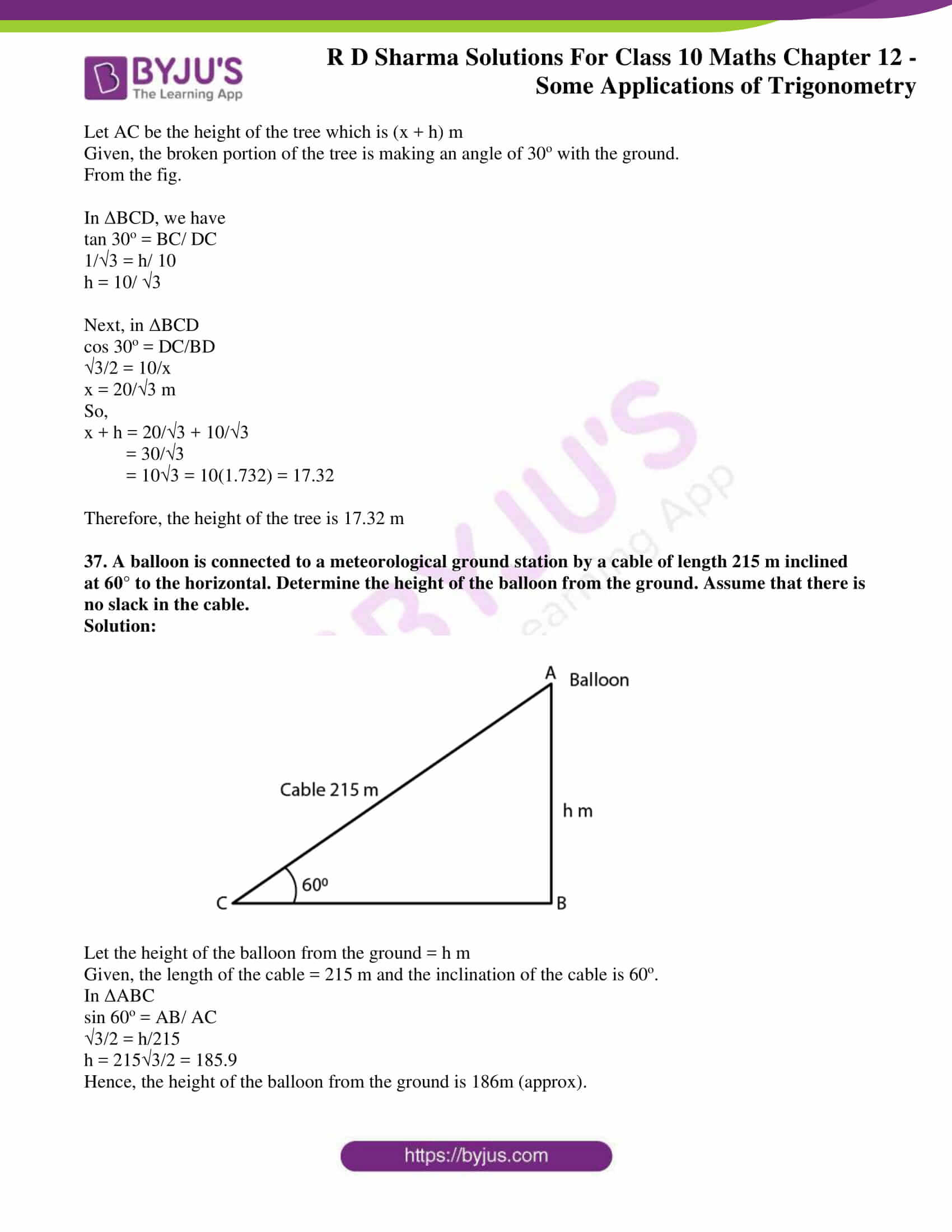Some Applications of Trigonometry is the 12th chapter of the RD Sharma Class 10 textbook. It contains only one exercise of problems based on using the trigonometric results to find heights and distances. For knowing the appropriate stepwise procedure and approach to these problems, the RD Sharma Solutions for Class 10 is the right place to dig in. All the solutions are developed based on the latest CBSE marking schemes.

### RD Sharma Class 10 Chapter 12 Exercise 12.1 Page No: 12.29

1. A tower stands vertically on the ground. From a point on the ground, 20 m away from the foot of the tower, the angle of elevation of the top the tower is 60°. What is the height of the tower?

Solution: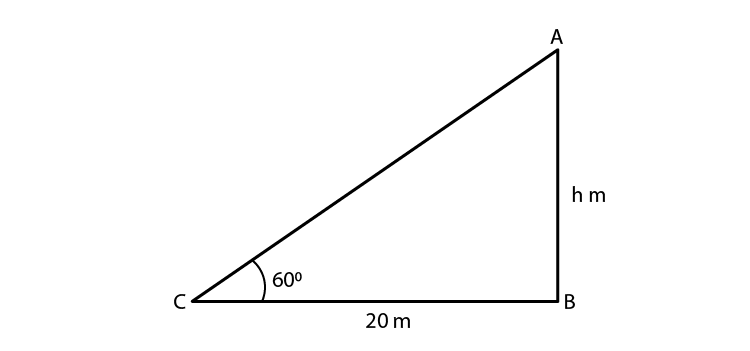Given:

Distance between the foot of the tower and point of observation = 20 m = BC

Angle of elevation of the top of the tower = 60° = θ

And, Height of tower (H) = AB

Now, from fig. ABC

ΔABC is a right angle triangle,

So,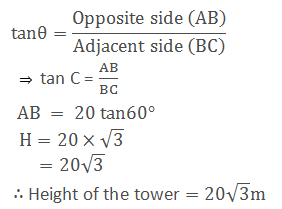2. The angle of elevation of a ladder against a wall is 60° and the foot of the ladder is 9.5 m away from the wall. Find the length of the ladder.

Solution:Given:

Distance between the wall and foot of the ladder = 9.5 m

Angle of elevation (θ) = 60°

Length of the ladder = L = AC

Now, from fig. ABC

ΔABC is a right angle triangle,

So,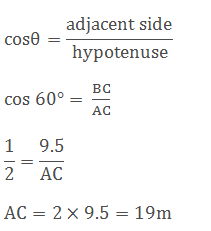Thus, length of the ladder (L) = 19 m

3. A ladder is placed along a wall of a house such that its upper end is touching the top of the wall. The foot of the ladder is 2 m away from the wall and the ladder is making an angle of 60° with the level of the ground. Determine the height of the wall.

Solution: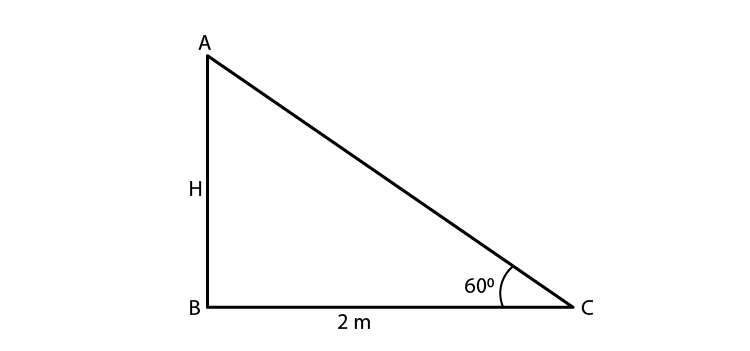Given,

Distance between the wall and the foot of the ladder = 2m = BC

Height of the wall (H) = AB

Now, the fig. of ABC forms a right angle triangle.

So,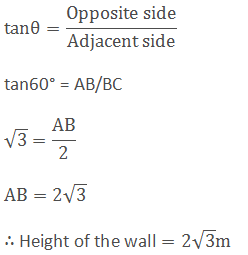4. An electric pole is 10 m high. A steel wire tied to top of the pole is affixed at a point on the ground to keep the pole up right. If the wire makes an angle of 45° with the horizontal through the foot of the pole, find the length of the wire.

Solution: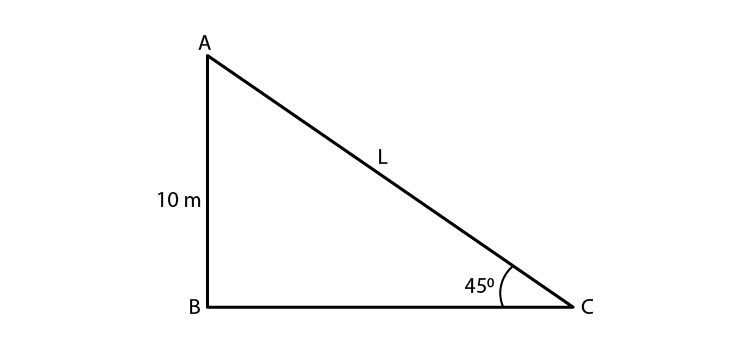Given,

Height of the electric pole = 10 m = AB

The angle made by steel wire with ground (horizontal) θ = 45°

Let length of wire = L = AC

So, form the fig. formed we have ABC as a right triangle.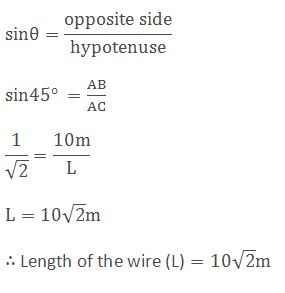5. A kite is flying at a height of 75 meters from the ground level, attached to a string inclined at 60° to the horizontal. Find the length of the string to the nearest meter.

Solution: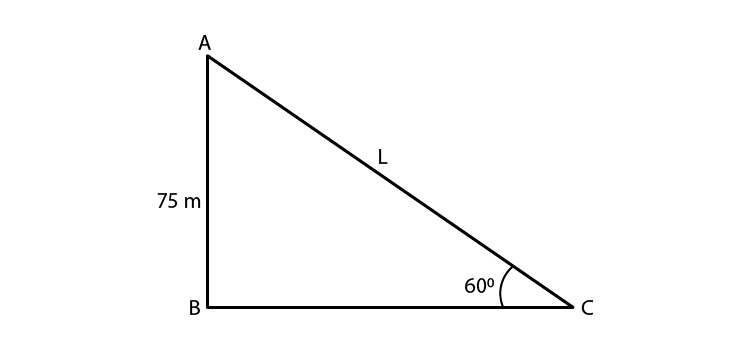Given,

Height of kite flying from the ground level = 75 m = AB

Angle of inclination of the string with the ground (θ) = 60°

Let the length of the string be L = AC

So, form the fig. formed we have ABC as a right triangle.

Hence,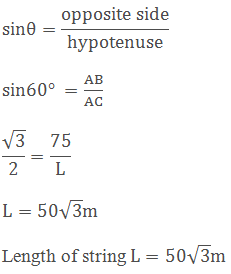6. A ladder 15 metres long reaches the top of a vertical wall. If the ladder makes an angle of 60o with the wall, find the height of the wall.

Solution: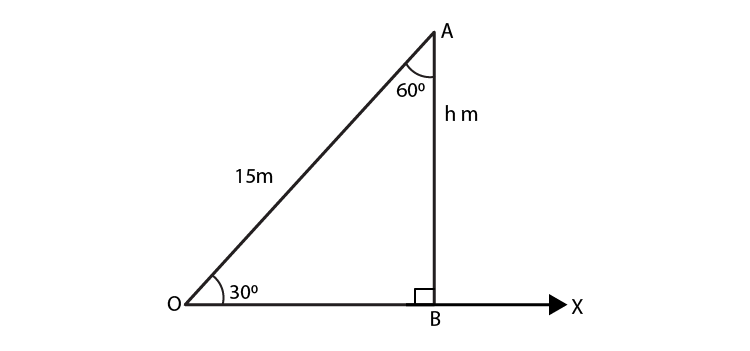Given,

The length of the ladder = 15m = AO

Let the height of the wall be h metres.

And the horizontal ground taken as OX.

Then from the fig. we have,

In right ΔABO, using trigonometric ratios

cos (60o) = AB/AO

1/2 = h/ 15

h = 15/2

h = 7.5m

Hence, the height of the wall is 7.5m

7. A vertical tower stands on a horizontal place and is surmounted by a vertical flag staff. At a point on the plane 70 meters away from the tower, an observer notices that the angles of elevation of the top and bottom of the flag-staff are respectively 60° and 45°. Find the height of the flag staff and that of the tower.

Solution: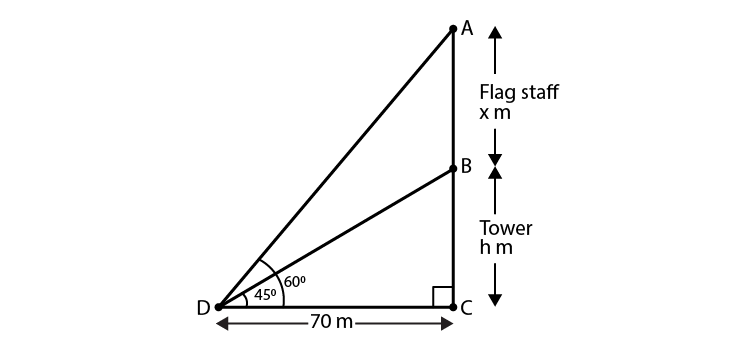Given,

A vertical tower is surmounted by flag staff.

Distance between observer and the tower = 70 m = BC

Angle of elevation of bottom of the flag staff = 45°

Angle of elevation of top of the flag staff = 60°

Let the height of the flag staff = h = AD

Height of tower = H = AB

If we represent the above data in the figure then it forms right angle triangles ΔABC and ΔBCD

When θ is angle in right angle triangle we know that

tan θ = opp. Side/ Adj. side

Now,

tan 45o = AB/ BC

1 = H/ 70

∴ H =70

Again,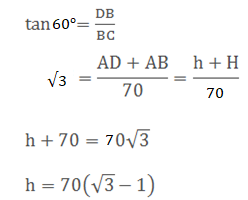h = 70 (1.732-1)

∴ h = 51.24 m

Therefore, the height of tower = 70 m and the height of flag staff = 51.24 m

8. A vertically straight tree, 15 m high, is broken by the wind in such a way that its top just touches the ground and makes an angle of 60° with the ground. At what height from the ground did the tree break?

Solution: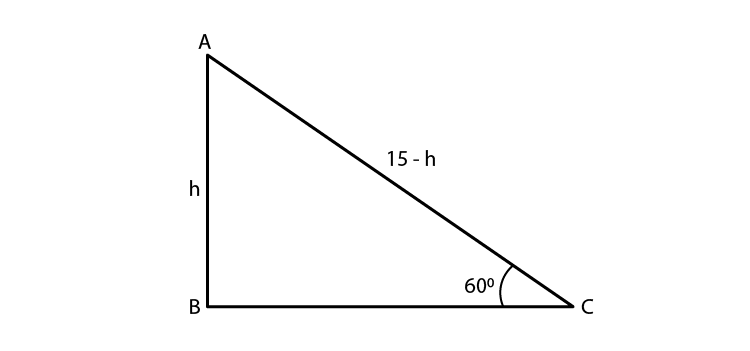Given,

The initial height of tree H = 15 m = AB + AC

Let us assume that it is broken at point A.

And, the angle made by broken part with the ground (θ) = 60°

Height from ground to broken points = h = AB

So, we have

H = AC + h

⟹ AC = (H – h) m

We get a right triangle formed by the above given data,

So,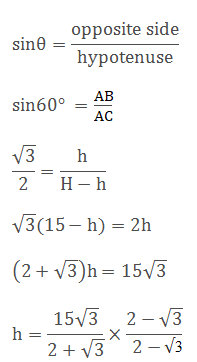Rationalizing denominator, we have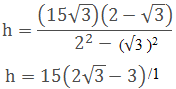Therefore, the height of broken point from the ground is 15(2√3 – 3)m

9. A vertical tower stands on a horizontal plane and is surmounted by a vertical flag staff of height 5 meters. At a point on the plane, the angles of elevation of the bottom and the top of the flag staff are respectively 30° and 60°. Find the height of the tower.

Solution: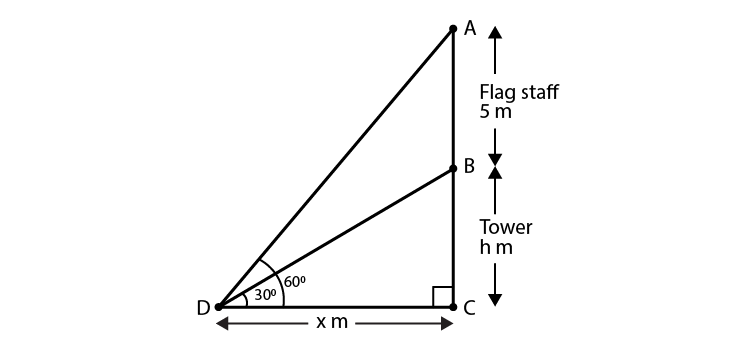Given,

Height of the flag staff = 5 m =AB

Angle of elevation of the top of flag staff = 60°

Angle of elevation of the bottom of the flagstaff = 30°

Let height of tower be ‘h’ m = BC

And, let the distance of the point from the base of the tower = x m

In right angle triangle BCD, we have

tan 30o = BC/DC

1/√3 = h/x

x = h√3 ….. (i)

Now, in ΔACD,

tan 60o = AC/DC

√3 = (5 + h)/ x

√3x = 5 + h

√3(h√3) = 5 + h [using (i)]

3h = 5 + h

2h = 5

h = 5/2 = 2.5m

Therefore, the height of the tower = 2.5 m

10. A person observed the angle of elevation of a tower as 30°. He walked 50 m towards the foot of the tower along level ground and found the angle of elevation of the top of the tower as 60°. Find the height of the tower.

Solution: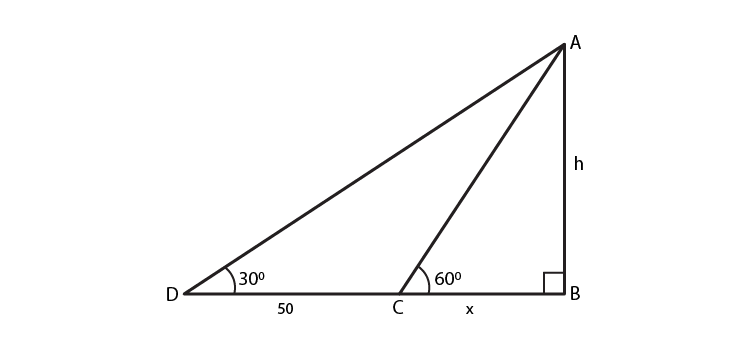Given,

The angle of elevation of the tower before he started walking = 30o

Distance walked by the person towards the tower = 50m

The angle of elevation of the tower after he walked = 60o

Let height of the tower (AB) = h m

Let the distance BC = x m

From the fig. in ΔABC,

tan 60o = AB/ BC

√3 = h/x

x = h/√3 ….(i)

Now, in ΔABD

tan 30o = AB/ BD

1/√3 = h/ (50 + x)

√3h = 50 + x

√3h = 50 + (h/√3) [using (i)]

3h = 50√3 + h

2h = 50√3

h = 25√3 = 25(1.73) = 43.25m

Therefore, the height of the tower = 43.25m

11. The shadow of a tower, when the angle of elevation of the sun is 45°, is found to be 10 m longer than when it was 60°. Find the height of the tower.

Solution: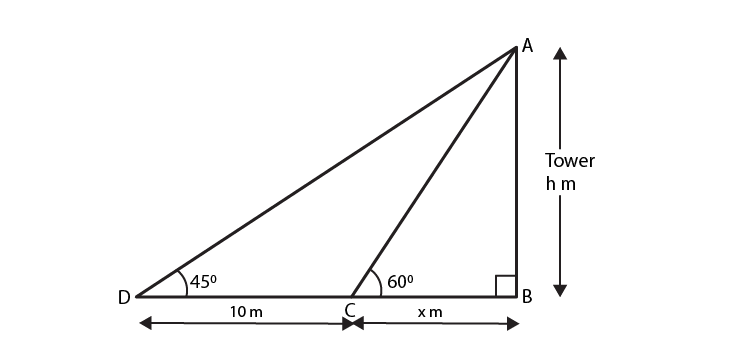Let the height of the tower(AB) = h m

Let the length of the shorter shadow be x m

Then, the longer shadow is (10 + x)m

So, from fig. In ΔABC

tan 60o = AB/BC

√3 = h/x

x = h/√3…. (i)

Next, in ΔABD

tan 45o = AB/BD

1 = h/(10 + x)

10 + x = h

10 + (h/√3) = h [using (i)]

10√3 + h = √3h

h(√3 -1) =10√3

h = 10√3/ (√3 -1)

After rationalising the denominator, we have

h = [10√3 x (√3 + 1)]/ (3 – 1)

h = 5√3(√3 + 1)

h = 5(3 + √3) = 23.66 [√3 = 1.732]

Therefore, the height of the tower is 23.66 m.

12. A parachute is descending vertically and makes angles of elevation of 45° and 60° at two observing points 100 m apart from each other on the left side of himself. Find the maximum height from which he falls and the distance of point where he falls on the ground from the just observation point.

Solution: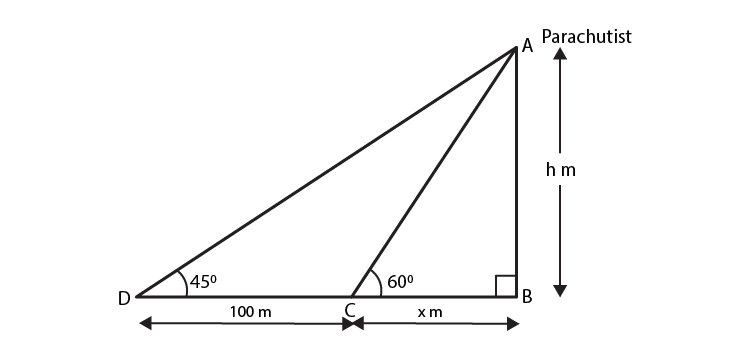Let the parachute at highest point A and let C and D be points which are 100 m apart on ground where from then CD = 100 m

Angle of elevation from point D = 45° = α

Angle of elevation from point C = 60° = β

Let B be the point just vertically down the parachute

Let us draw figure according to above data then it forms the figure as shown in which ABC and ABD are two triangles

Maximum height of the parachute from the ground

AB = h m

Distance of point where parachute falls to just nearest observation point = x m

If in right angle triangle one of the included angles is θ then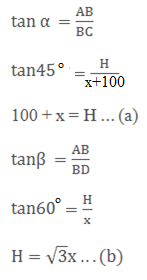From (a) and (b)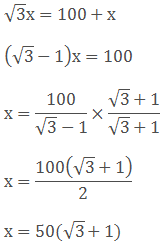x = 136.6 m in (b)

h = √3 × 136.6 = 236.6m

Therefore,

The maximum height of the parachute from the ground, H = 236.6m

Distance between the two points where parachute falls on the ground and just the observation is x = 136.6 m

13. On the same side of a tower, two objects are located. When observed from the top of the tower, their angles of depression are 45° and 60°. If the height of the tower is 150 m, find the distance between the objects.

Solution: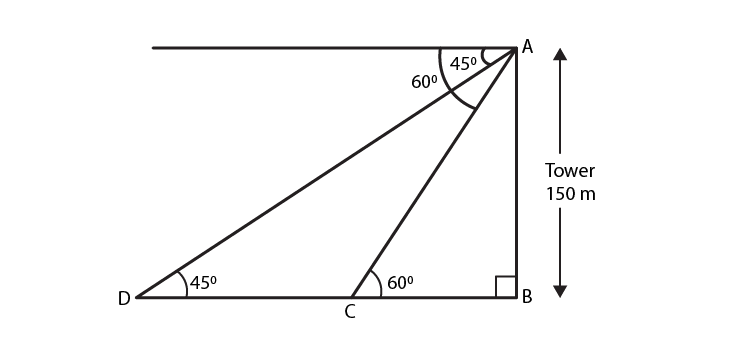Given,

The height of the tower (AB) = 150m

Angles of depressions of the two objects are 45o and 60o.

In ΔABD

tan 45o = AB/ BD

1 = 150/ BD

BD = 150m

Next, in ΔABC

tan 60o = AB/ BC

√3 = 150/ BC

BC = 150√3/ 3

BC = 50√3 = 50(1.732) = 86.6 m

So,

DC = BD – BC = 150 – 86.6 = = 63.4

Therefore, the distance between two objects = 63.4 m

14. The angle of elevation of a tower from a point on the same level as the foot of the tower is 30°. On advancing 150 meters towards the foot of the tower, the angle of elevation of the tower becomes 60°. Show that the height of the tower is 129.9 metres.

Solution: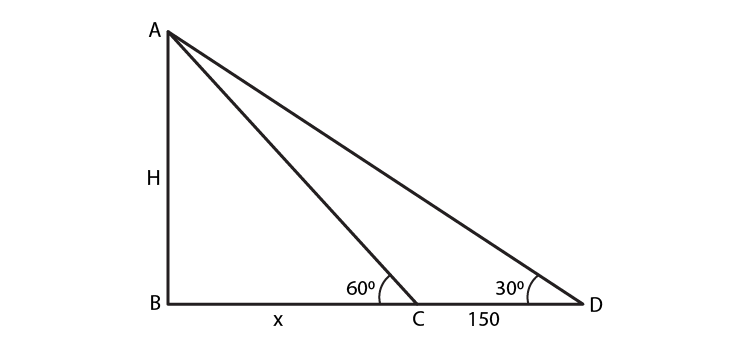Given,

The angle of elevation of top tower from first point D, α = 30°

On advancing through D to C by 150 m, then CD = 150 m

Angle of elevation of top of the tower from second point C, β = 60°

Let height of tower AB = H m

Representing the above data in form of figure then it form a figure as shown with ∠B = 90°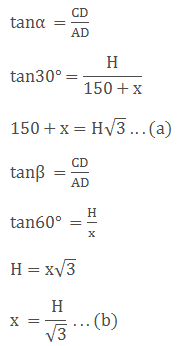Substituting (b) in (a), we have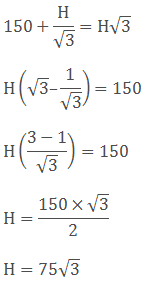H = 129.9

Therefore, the height of the tower = 129.9 m

15. The angle of elevation of the top of a tower as observed from a point in a horizontal plane through the foot of the tower is 32°. When the observer moves towards the tower a distance of 100 m, he finds the angle of elevation of the top to be 63°. Find the height of the tower and the distance of the first position from the tower. [Take tan 32° = 0.6248 and tan 63° = 1.9626]

Solution: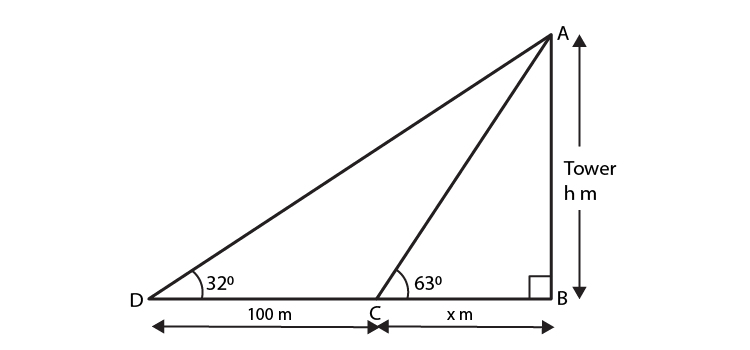Let the height of the tower = h m

And the distance BC = x m

Then, from the fig.

In ΔABC

tan 63o = AB/BC

1.9626 = h/x

x = h/ 1.9626

x = 0.5095 h …. (i)

Next, in ΔABD

tan 32o = AB/ BD

0.6248 = h/ (100 + x)

h = 0.6248(100 + x)

h = 62.48 + 0.6248x

h = 62.48 + 0.6248(0.5095 h) ….. [using (i)]

h = 62.48 + 0.3183h

0.6817h = 62.48

h = 62.48/0.6817 = 91.65

Using h in (i), we have

x = 0.5095(91.65) = 46.69

Therefore,

the height of the tower is 91.65 m

Distance of the first position from the tower = 100 + x = 146.69 m

16. The angle of elevation of the top of a tower from a point A on the ground is 30°. On moving a distance of 20 meters towards the foot of the tower to a point B the angle of elevation increases to 60°. Find the height of the tower and the distance of the tower from the point A.

Solution: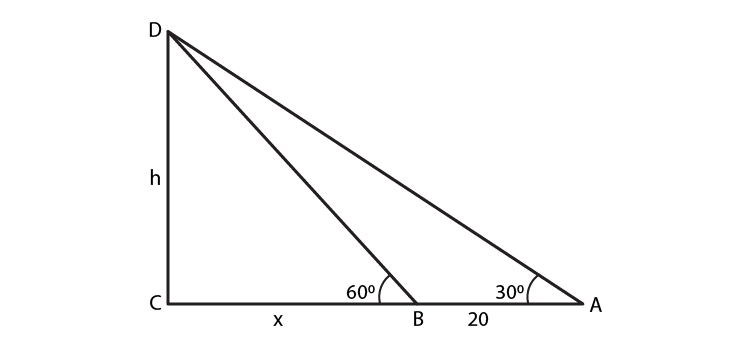Given,

Angle of elevation of top of the tower from point A, α = 30°.

Angle of elevation of top of tower from point B, β = 60°.

And, the distance between A and B, AB = 20 m

Let height of tower CD = ‘h’ m and distance between second point B from foot of the tower be ‘x’ m

Representing the above data in form of figure with ∠D = 90°,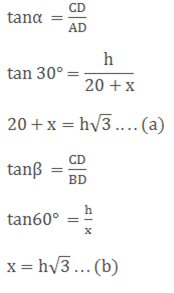Substituting (b) in (a), we have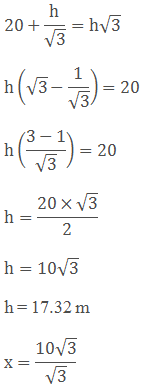x = 10 m

Therefore,

Height of the tower = 17.32 m

Distance of the tower from point A = (20 + 10) = 30 m

17. From the top of a building 15 m high the angle of elevation of the top of tower is found to be 30°. From the bottom of the same building, the angle of elevation of the top of the tower is found to be 60°. Find the height of the tower and the distance between the tower and the building.

Solution: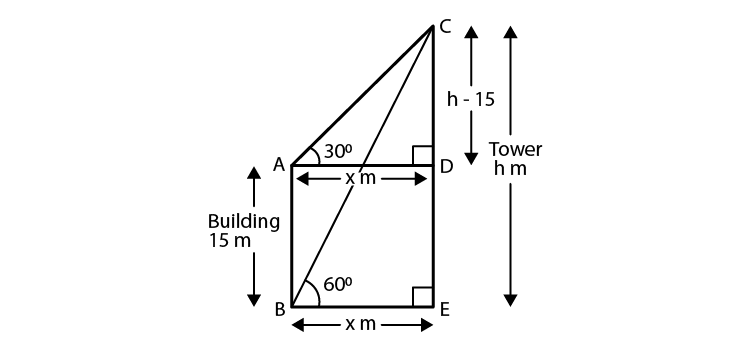Given,

The height of the building = 15 m

The angle of elevation from the top of the building to top of the tower = 30o

The angle of elevation from the bottom of the building to top of the tower = 60o

Let the height of the tower = h m

And the distance between tower and building = x m

Similarly, AB = DE

Then, from fig.

In ΔBEC,

tan 60o = CE/BE

√3 = h/x

x = h/√3 ….(i)

Next, in ΔCDA,

tan 30o = CD/DA

1/ √3 = (h – 15)/x

√3h – 15√3 = x

√3h – 15√3 = h/√3 [using (i)]

3h – 45 = h

2h = 45

h = 45/2 = 22.5

Putting h in (i), we get

x = 22.5/(√3)

x = 22.5/(1.73) = 12.975

Therefore, the height of the building = 22.5 m

And, distance between tower and building = 12.975 m

18. On a horizontal plane there is a vertical tower with a flag pole on the top of the tower. At a point 9 m away from the foot of the tower the angle of elevation of the top and bottom of the flag pole are 60° and 30° respectively. Find the height of the tower and the flag pole mounted on it.

Solution: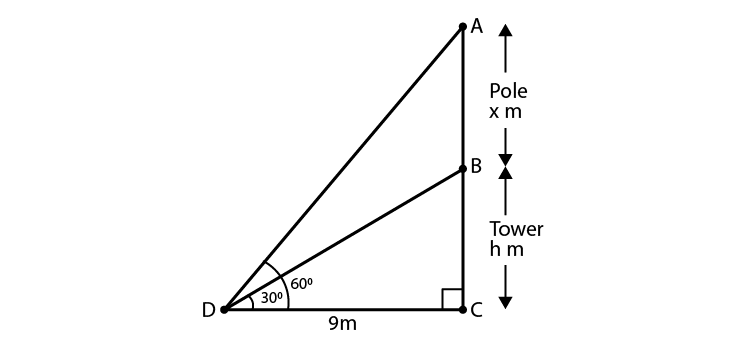Let BC be the tower and AB be the flagstaff on the tower

Distance of the point of observation from foot of the tower DC = 9 m

Angle of elevation of top of flagstaff is 60°

Angle of elevation of bottom of flagstaff is 30°

Let height of the tower = h m = BC

Height of the pole = x m = AB

From fig, we have

In ΔBCD,

tan 30o = BC/DC

1/√3 = h/9

h = 9/√3 = 3√3

Next, in ΔACD

tan 60o = AC/DC

√3 = (x + h)/9

x + h = 9√3

x + 3√3 = 9√3

x = 6√3 m

Therefore,

Height of the tower = 3√3 m

And, height of the pole = 6√3 m

19. A tree breaks due to storm and the broken part bends so that the top of the tree touches the ground making an angle of 30° with the ground. The distance between the foot of the tree to the point where the top touches the ground is 8 m. Find the height of the tree.

Solution: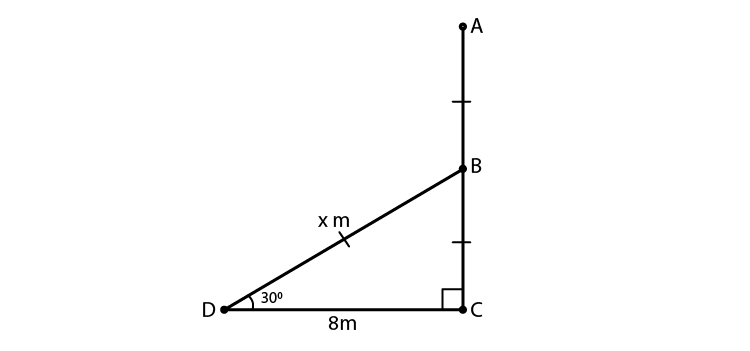Let the initial height of the tree be AC.

And, due to storm the tree is broken at B.

Let the bent portion of the tree be AB = x m and the remaining portion BC = h m

So, the height of the tree AC = (x + h) m

And, given DC = 8m

Now, in ΔBCD

tan 30o = BC/DC

1/√3 = h/8

h = 8/√3

Next, in ΔBCD

cos 30o = DC/BD

√3/2 = 8/x

x = 16/√3 m

So, x + h = 16/√3 + 8/√3

= 24/√3 = 8√3

Therefore, the height of the tree is 8√3 m.

20. From a point P on the ground the angle of elevation of a 10 m tall building is 30°. A flag is hoisted at the top of the building and the angle of elevation of the top of the flag staff from P is 45°. Find the length of the flag staff and the distance of the building from the point P.

Solution: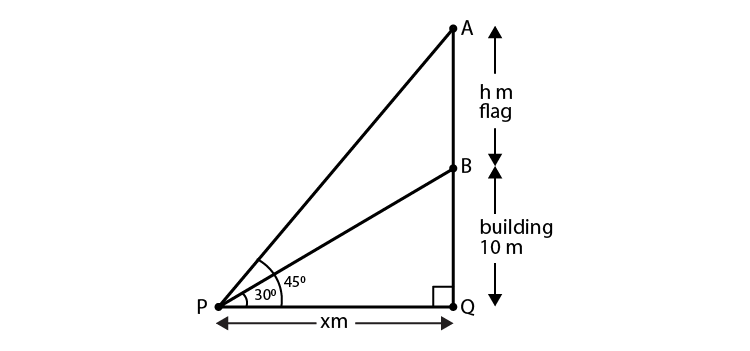Let the height of flag-staff(AB) = h m

And, the distance PQ = x m

Given,

Angle of elevation of top of the building = 30o

Angle of elevation of top of the flag staff = 45o

From the fig.

In ΔBQP,

tan 30o = BQ/PQ

1/√3 = 10/x

x = 10√3 m

Next,

In ΔAQP,

tan 45o = AQ/PQ

1 = (h + 10)/x

h + 10 = x = 10√3

h = (10√3 – 10) = 10(1.732) – 10 = 17.32 – 10

= 7.32 m

Therefore, the distance of point P from building = x = 10√3 = 10(1.732) = 17.32m

21. A 1.6 m tall girl stands at a distance of 3.2 m from a lamp post and casts a shadow of 4.8 m on the ground. Find the height of the lamp post by using (i) trigonometric ratio (ii) properties of similar triangles.

Solution: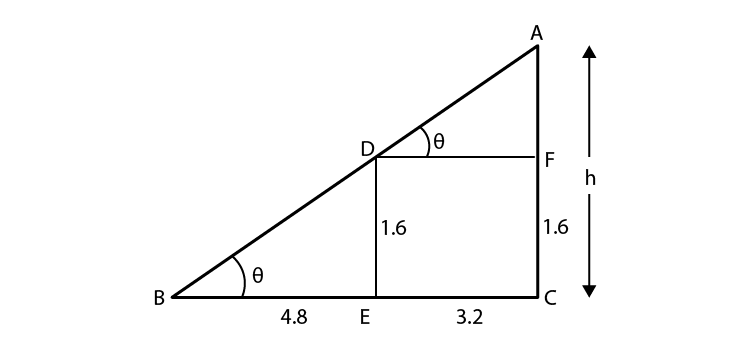Let AC be the lamp post of height ‘h’

DE is the tall girl and her shadow is BE.

So, we have ED = 1.6 m, BE = 4.8 m and EC = 3.2

(i) By using trigonometric ratio

In ΔBDE,

tan θ = 1.6/4.8

tan θ = 1/3

Next, In ΔABC

tan θ = h/ (4.8 + 3.2)

1/3 = h/8

h = 8/3 m

(ii) By using similar triangles

Since triangle BDE and triangle ABC are similar (by AA criteria), we have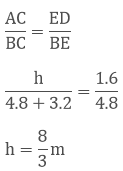Therefore, the height of the lamp post is h = 8/3 m

22. A 1.5 m tall boy is standing at some distance from a 30 m tall building. The angle of elevation from his eyes to the top of the building increases from 30° to 60° as he walks towards the building. Find the distance he walked towards the building.

Solution: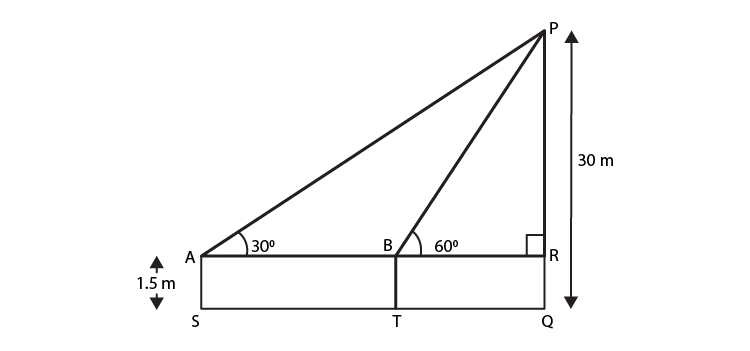Given,

The height of the tall boy (AS) = 1.5 m

The length of the building (PQ) = 30 m

Let the initial position of the boy be S. And, then he walks towards the building and reached at the point T.

From the fig. we have

AS = BT = RQ = 1.5 m

PR = PQ – RQ = (30 – 1.5)m = 28.5 m

In ΔPAR,

tan 30o = PR/AR

1/ √3 = 28.5/ AR

AR = 28.5√3

In ΔPRB,

tan 60o = PR/BR

√3 = 28.5/ BR

BR = 28.5/√3 = 9.5√3

So, ST = AB = AR – BR = 28.5√3 – 9.5√3 = 19√3

Therefore, the distance which the boy walked towards the building is 19√3 m.

23. The shadow of a tower standing on level ground is found to be 40 m longer when Sun’s altitude is 30° than when it was 60°. Find the height of the tower.

Solution: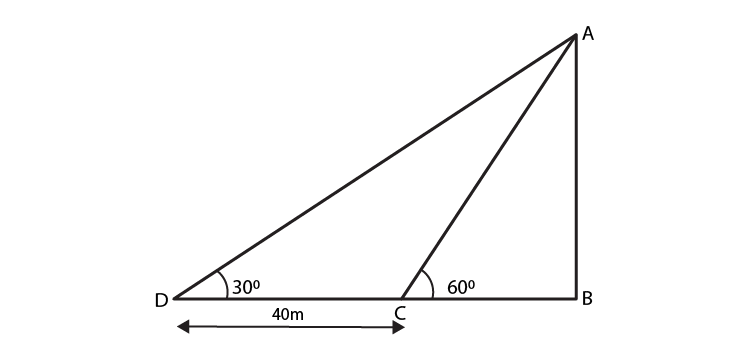When the sun’s altitude is the angle of elevation of the top of the tower from the tip of the shadow.

Let AB be h m and BC be x m. From the question, DB is 40 m longer than BC.

So, BD = (40 + x) m

And two right triangles ABC and ABD are formed.

In ΔABC,

tan 60o = AB/ BC

√3 = h/x

x = h/√3 … (i)

In ΔABD,

tan 30o = AB/ BD

1/ √3 = h/ (x + 40)

x + 40 = √3h

h/√3 + 40 = √3h [using (i)]

h + 40√3 = 3h

2h = 40√3

h = 20√3

Therefore, the height of the tower is 20√3 m.

24. From a point on the ground the angle of elevation of the bottom and top of a transmission tower fixed at the top of 20 m high building are 45° and 60° respectively. Find the height of the transmission tower.

Solution: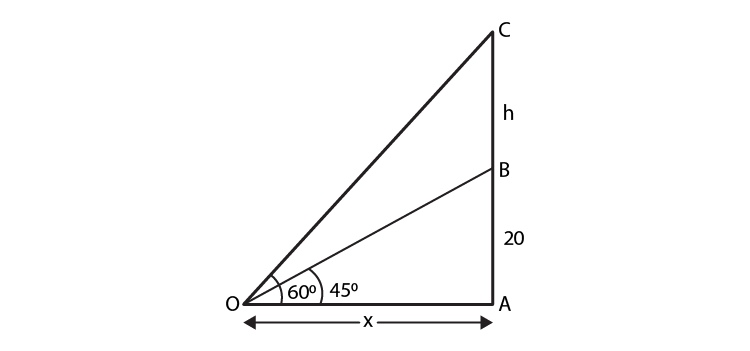Given,

Height of the building = 20 m = AB

Let height of tower above building = h = BC

Height of tower + building = (h + 20) m [from ground] = CA

Angle of elevation of bottom of tower, α = 45°

Angle of elevation of top of tower, β = 60°

Let distance between tower and observation point = x m

Then, from the fig. we have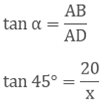x = 20 m

Next,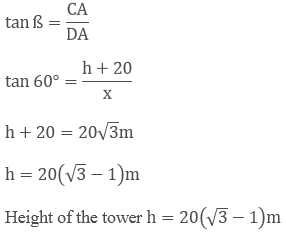h = 20(1.73 − 1) = 20 x .732 = 14.64

Therefore, the height of the tower is 14.64m

25. The angle of depression of the top and bottom of 8 m tall building from the top of a multistoried building are 30° and 45°. Find the height of the multistoried building and the distance between the two buildings.

Solution: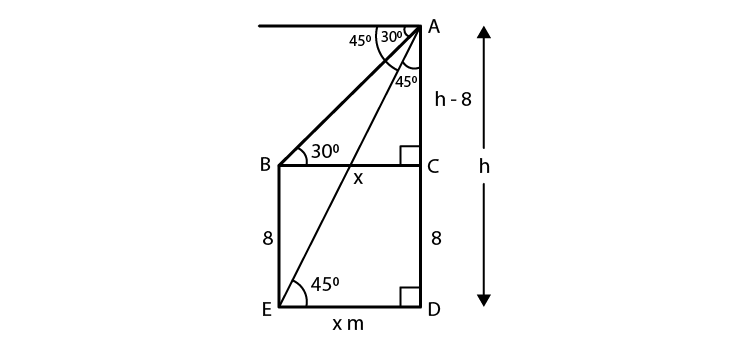Let height of multistoried building ‘h’ m = AD

Height of the tall building = 8 m = BE

Angle of depression of top of the tall building from the multistoried building = 30°

Angle of depression of bottom of the tall building from the multistoried building = 30°

And, let the distance between the two buildings = ‘x’ m = ED

So, BC = x and CD = 8 m [As BCDE forms a rectangle]

So, AC = (h – 8) m

From the fig. we have

In ΔBCA

tan 30o = AC/ BC

1/√3 = (h – 8)/x

x = √3(h – 8)…… (i)

1 = h/ x

h = x

h = √3(h – 8) [using (i)]

h = √3h – 8√3

(√3 – 1)h = 8√3

h = 8√3/ (√3 -1)

Rationalising the denominator by (√3 + 1), we have

h = 8√3(√3 + 1)/ (3 – 1)

h = 4√3(√3 + 1)

h = 12 + 4√3

= 4(3 + √3)

Thus, x = h = 4(3 + √3)

Therefore, the height of the building is 4(3 + √3)m and the distance between the building is also 4(3 + √3)m

26. A statue 1.6 m tall stands on the top of a pedestal. From a point on the ground, angle of elevation of the top of the statue is 60° and from the same point the angle of elevation of the top of the pedestal is 45°. Find the height of the pedestal.

Solution: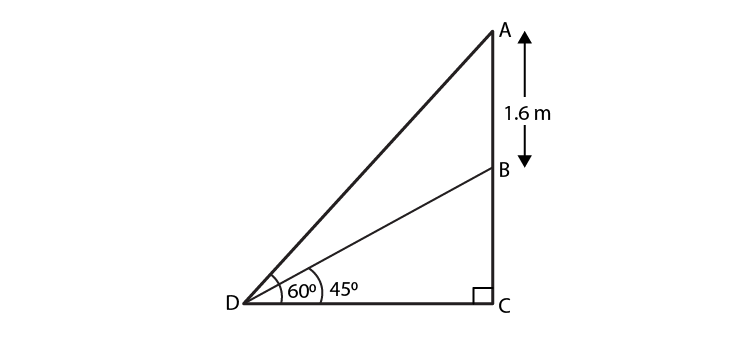Let’s assume AB as the statue, BC be the pedestal and D be the point on ground from where eleveation angles are measured.

Given,

Angle of elevation of the top of statue is 60o

Angle of elevation of the top of the pedestal is 45o

So, from the fig. we have

In ΔBCD,

tan 45o = BC/ CD

1 = BC/ CD

CD = BC

tan 60o = (AB + BC)/ CD

√3 = (AB + BC)/ BC [As CD = BC, found above]

BC√3 = AB + BC

AB = (√3 – 1)BC

BC = AB/ (√3 – 1)

BC = 1.6/ (√3 – 1)

Rationalising the denominator, we have

BC = 1.6(√3 + 1)/ 2

BC = 0.8(√3 + 1) m

Therefore, the height of pedestal is 0.8(√3 + 1) m

27. A T.V. tower stands vertically on a bank of a river of a river. From a point on the other bank directly opposite the tower, the angle of elevation of the top of the tower is 60°. From a point 20 m away this point on the same bank, the angle of elevation of the top of the tower is 30°. Find the height of the tower and the width of the river.

Solution: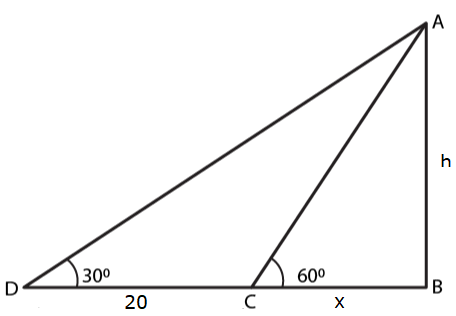Let AB be the T.V tower of height ‘h’ m on the bank of river and ‘D’ be the point on the opposite side of the river. An angle of elevation at the top of the tower is 30°

Let AB = h and BC = x

And, given CD = 20 m

From fig. we have

In ΔACB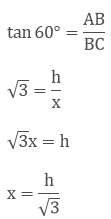Next, in ΔDBA,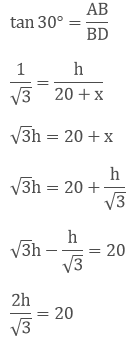h = 10√3 m

And,

x = h/√3

x = 10√3/ √3

x = 10

Therefore, the height of the tower is 10√3 m and width of the river is 10 m.

28. From the top of a 7 m high building, the angle of elevation of the top of a cable is 60° and the angel of depression of its foot is 45°. Determine the height of the tower.

Solution: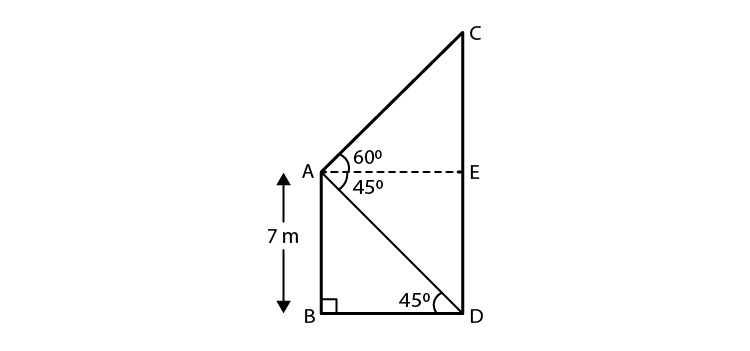Given

Height of the building = 7 m = AB

Height of the cable tower = CD

Angle of elevation of the top of the cable tower from the top of the building = 60°

Angle of depression of the bottom of the building from the top of the building= 45°

Then, from the fig. we see that

ED = AB = 7 m

And,

CD = CE + ED

So, In ΔABD, we have

AB/ BD = tan 45o

AB = BD = 7

BD = 7

In ΔACE,

AE = BD = 7

And, tan 60o = CE/AE

√3 = CE/ 7

CE = 7√3 m

So, CD = CE + ED = (7√3 + 7)= 7(√3 + 1) m

Therefore, the height of the cable tower is 7(√3 + 1)m

29. As observed from the top of a 75 m tall lighthouse, the angles of depression of two ships are 30° and 45°. If one ship is exactly behind the other on the same side of the lighthouse, find the distance between the two ships.

Solution: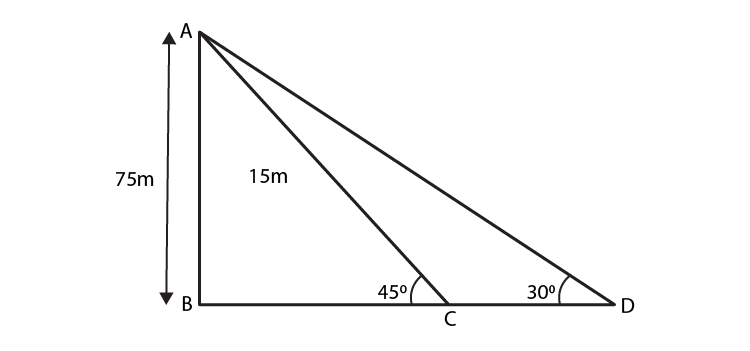Given;

Height of the lighthouse = 75m = ‘h’ m = AB

Angle of depression of ship 1, α = 30°

Angle of depression of bottom of the tall building, β = 45°

The above data is represented in form of figure as shown

Let distance between ships be ‘x’ m = CD

If in right angle triangle one of the included angle is θ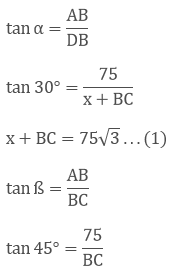BC = 75 … (2)

Substituting (2) in (1)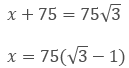Therefore, the distance from the ships is 75(√3 + 1) m

30. The angle of elevation of the top of the building from the foot of the tower is 30° and the angle of the top of the tower from the foot of the building is 60°. If the tower is 50 m high, find the height of the building.

Solution: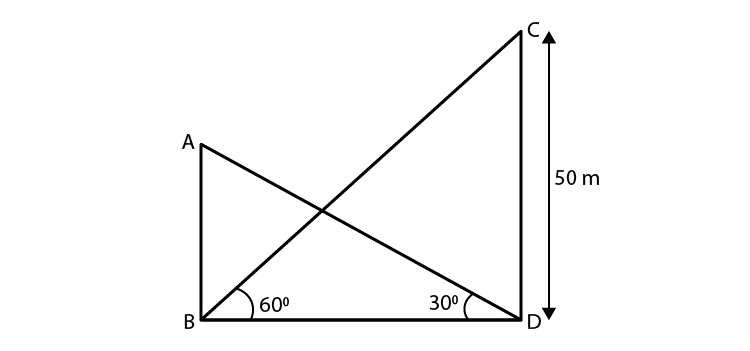Let AB be the building and CD be the tower.

Given,

The angle of elevation of the top of the building from the foot of the tower is 30o.

And, the angle of elevation of the top of the tower from the foot of the building is 60o.

Height of the tower = CD = 50 m

From the fig. we have

In ΔCDB,

CD/ BD = tan 60o

50/ BD = √3

BD = 50/√3 …. (i)

Next in ΔABD,

AB/ BD = tan 30o

AB/ BD = 1/√3

AB = BD/ √3

AB = 50/√3/ (√3) [From (i)]

AB = 50/3

Therefore, the height of the building is 50/3 m.

31. From a point on a bridge across a river the angle of depression of the banks on opposite side of the river are 30° and 45° respectively. If the bridge is at the height of 30 m from the banks, find the width of the river.

Solution: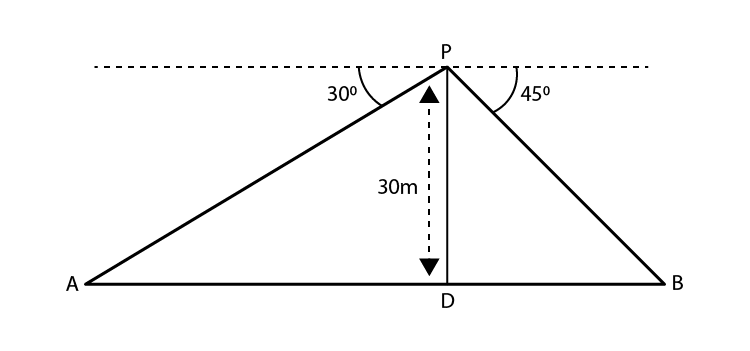Given,

The bridge is at a height of 30 m from the banks.

Let, A and B represent the points on the bank on opposite sides of the river. And, AB is the width of the river. P is a point on the bridge which is at the height of 30 m from the banks.

Now, from the fig, we have

In right ΔAPD,

∠A = 30o

So, tan 30o = PD/ AD

Next, in right ΔPBD

∠B = 45o

So, tan 45o = PD/ BD

1 = PD/ BD

BD = PD

BD = 30 m

We know that, AB = AD + DB = 30√3 + 30 = 30(√3 + 1)

Hence, the width of the river = 30(1 + √3)m

32. Two poles of equal heights are standing opposite to each other on either side of the road which is 80 m wide. From a point between them on the road the angles of elevation of the top of the poles are 60° and 30° respectively. Find the height of the poles and the distances of the point from the poles.

Solution: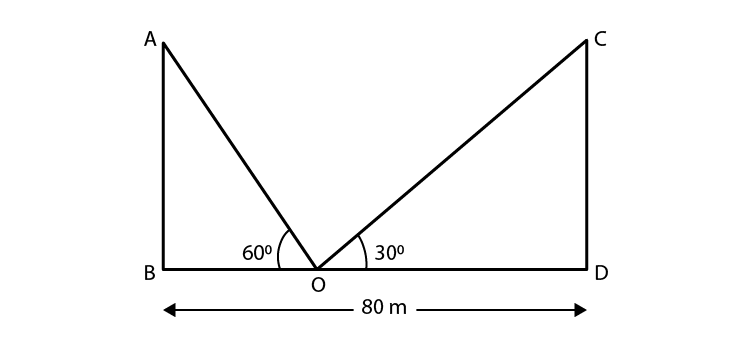Given,

Distance between the poles = 80 m = BD

Let the point of observation of the angles be O.

The angles of elevation to the top of the points is 60o and 30o

Let AB and CD be the poles and O is the point on the road.

From the fig. we have

In ΔAPD,

AB/ BO = tan 60o

AB/ BO = √3

BO = AB/ √3 ….(i)

Next in ΔCDO,

CD/ DO = tan 30o

CD/ (80 – BO) = 1/ √3

√3CD = 80 – BO

√3AB = 80 – (AB/√3) [As AB = CD and using (i)]

3AB = 80√3 – AB

4AB = 80√3

AB = 20√3

So, BO = 20√3/(√3) = 20 m

And, DO = BD – BO = 80 – 20 = 60

Hence, the height of the poles is 20√3 m and the point between the poles is 20m and 60 m far from these poles.

33. A man sitting at a height of 20 m on a tall tree on a small island in the middle of a river observes two poles directly opposite to each other on the two banks of the river and in line with foot of tree. If the angles of depression of the feet of the poles from a point at which the man is sitting on the tree on either side of the river are 60° and 30° respectively. Find the width of the river.

Solution: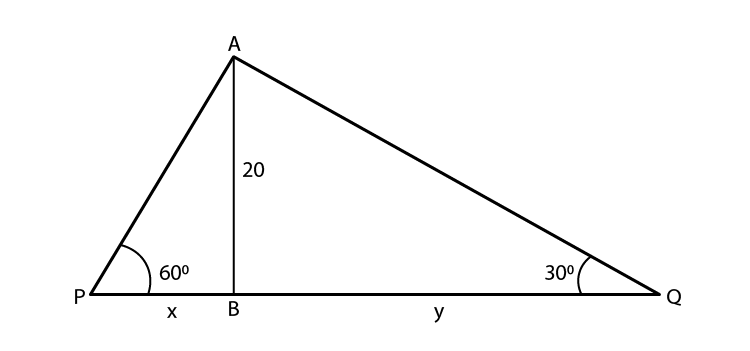From the given data, the fig. is made

Let width of river = PQ = (x + y) m

Height of tree (AB) = 20 m

So, in ΔABP

tan 60o = AB/ BP

√3 = 20/ x

x = 20/ √3 m

In ΔABQ,

tan 30o = AB/ BQ

1/ √3 = 20/ y

y = 20√3

So, (x + y) = 20/ √3 + 20√3

= [20 + 20(3)]/ √3

= 80/√3

Therefore, the width of the river is 80/√3 m.

34. A vertical tower stands on a horizontal plane and is surmounted by a flag staff of height 7m. From a point on the plane, the angle of elevation of the bottom of flag staff is 30° and that of the top of the flag staff is 45°. Find the height of the tower.

Solution: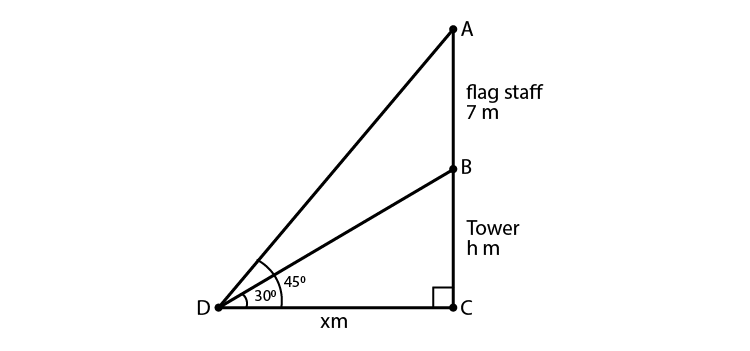Given,

The length of the flag staff = 7 m

Angles of elevation of the top and bottom of the flag staff from point D is 45o and 30o respectively.

Let the height of tower (BC) = h m

And, let DC = x m

So, in ΔABP

tan 30o = BC/DC

1/ √3 = h/ x

x = h√3…. (i)

And, in ΔACD

tan 45o = AC/ DC

1 = (7 + h)/ x

x = 7 + h

h√3 = 7 + h [from (i)]

h(√3 – 1) = 7

h = 7/(√3 – 1)

Now, rationalising the denominator we get

h = 7(√3 + 1)/ 2 = 7(1.732 + 1)/2 = 9.562

Therefore, the height of the tower is 9.56 m

35. The length of the shadow of a tower standing on level plane is found to be 2x meters longer when the sun’s attitude is 30° than when it was 30°. Prove that the height of tower is x(√3+1) meters.

Solution: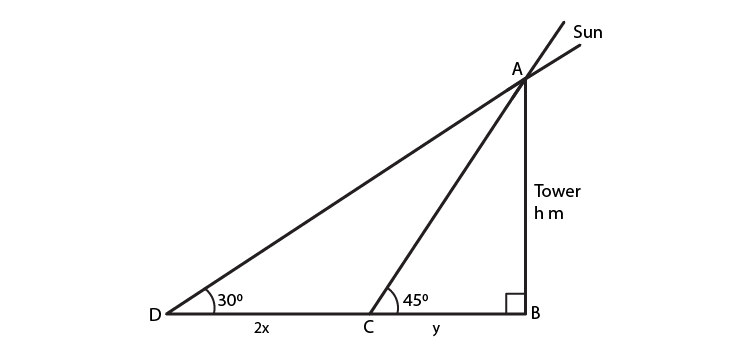From the question, the following fig. is made

Let the height of tower (AB) = h m

Let the distance BC = y m

Then, in ΔABC

tan 45o = AB/BC

1 = h/y

y = h

Next, in ΔABD

tan 30o = AB/BD

1/√3 = h/ (2x + y)

2x + y = √3h

2x + h = √3h

2x = (√3 – 1)h

h = 2x/ (√3 – 1)

Therefore, the height of the tower is 2x (√3 -1) m

• Hence Proved

36. A tree breaks due to storm and the broken part bends so that the top of the tree touches the ground making an angle of 30° with the ground. The distance from the foot of the tree to the point where the top touches the ground is 10 meters. Find the height of the tree.

Solution: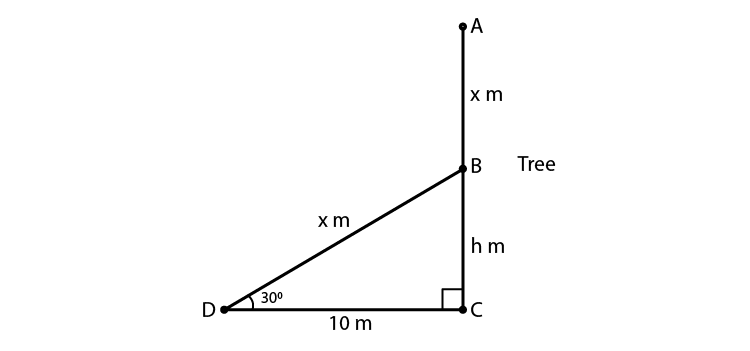Let AC be the height of the tree which is (x + h) m

Given, the broken portion of the tree is making an angle of 30o with the ground.

From the fig.

In ΔBCD, we have

tan 30o = BC/ DC

1/√3 = h/ 10

h = 10/ √3

Next, in ΔBCD

cos 30o = DC/BD

√3/2 = 10/x

x = 20/√3 m

So,

x + h = 20/√3 + 10/√3

= 30/√3

= 10√3 = 10(1.732) = 17.32

Therefore, the height of the tree is 17.32 m

37. A balloon is connected to a meteorological ground station by a cable of length 215 m inclined at 60° to the horizontal. Determine the height of the balloon from the ground. Assume that there is no slack in the cable.

Solution: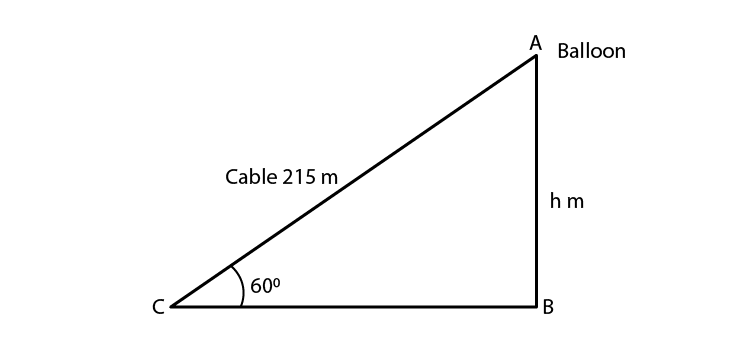Let the height of the balloon from the ground = h m

Given, the length of the cable = 215 m and the inclination of the cable is 60o.

In ΔABC

sin 60o = AB/ AC

√3/2 = h/215

h = 215√3/2 = 185.9

Hence, the height of the balloon from the ground is 186m (approx).